# RS Aggarwal Solutions for Class 8 Maths Chapter 3 - Square and Square Roots

RS Aggarwal Solutions For Class 8 Maths Chapter 3 Squares and Square Roots are provided here. You can download the pdf of RS Aggarwal Solutions for Class 8 Maths Chapter 3 Squares and Square Roots from the given links. Class 8 is an important phase of a student’s life. It is crucial concepts which are taught in Class 8 are understood thoroughly as these concepts are continued in Class 9 and 10 and are also the foundation for higher studies. Here we will learn about different methods of calculating the square root of numbers, properties of perfect squares, calculating square roots for fractions as well as decimals.

If you want to score good marks in class 8 Mathematics examination, it is advised to practice the RS Aggarwal Solutions for Class 8. Therefore, we at BYJU’S provide answers to all questions uniquely and briefly. Now learn this topic in depth with the RS Aggarwal Class 8 Solutions with step by step illustrations, which will make learning easy for students.

## Download pdf of RS Aggarwal Solutions For Class 8 Maths Chapter 3 Squares and Square Roots

### Access answers to Maths RS Aggarwal Solutions for Class 8 Chapter 3 – Squares and Square Roots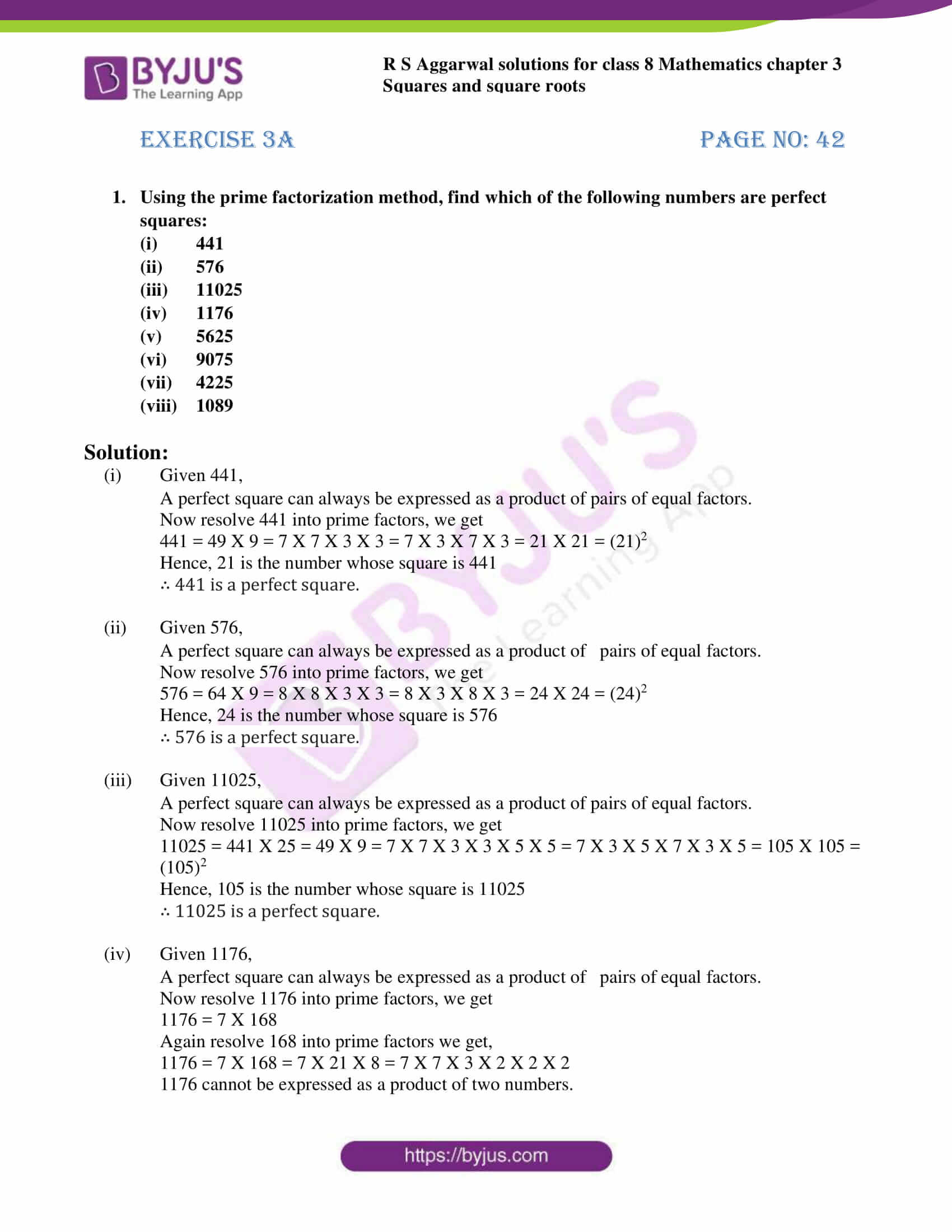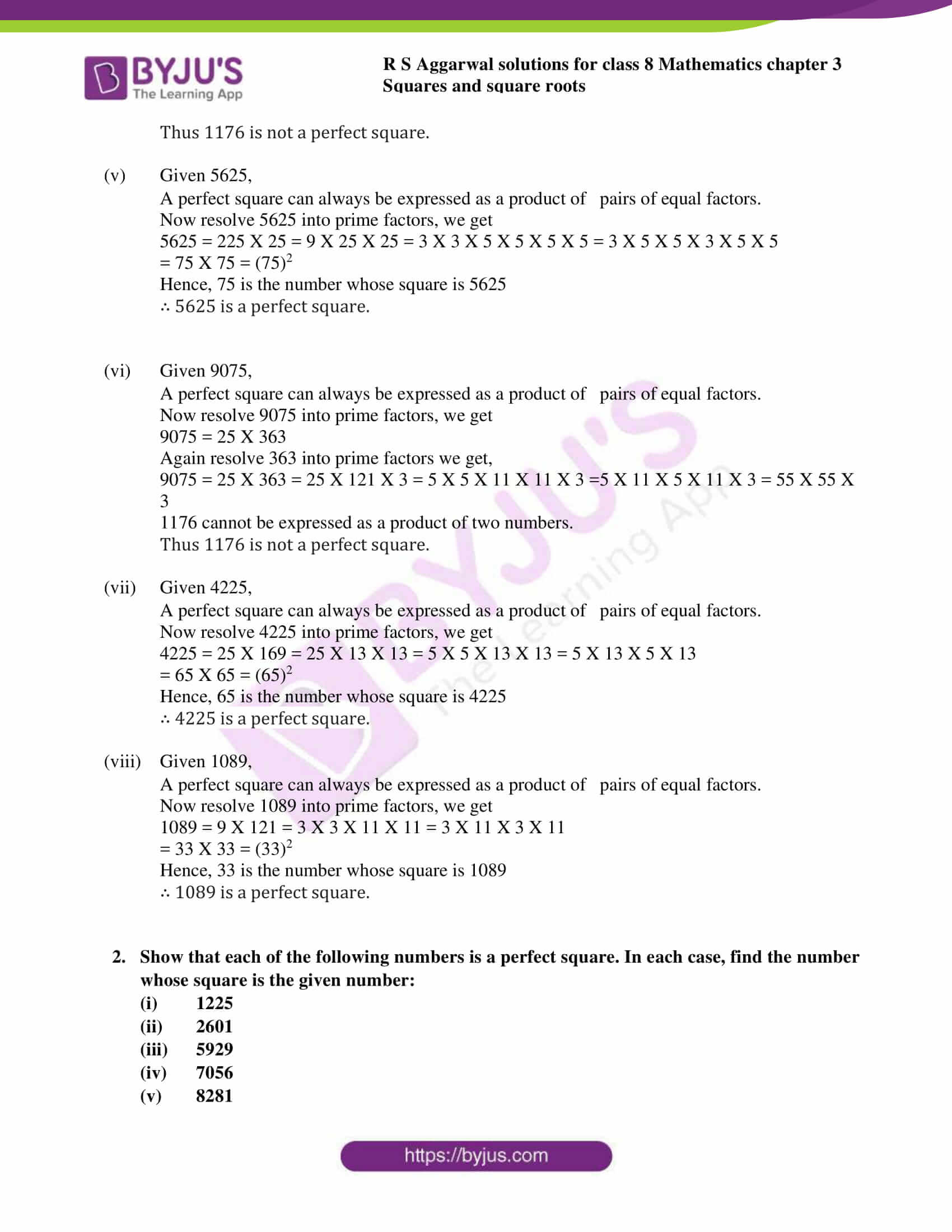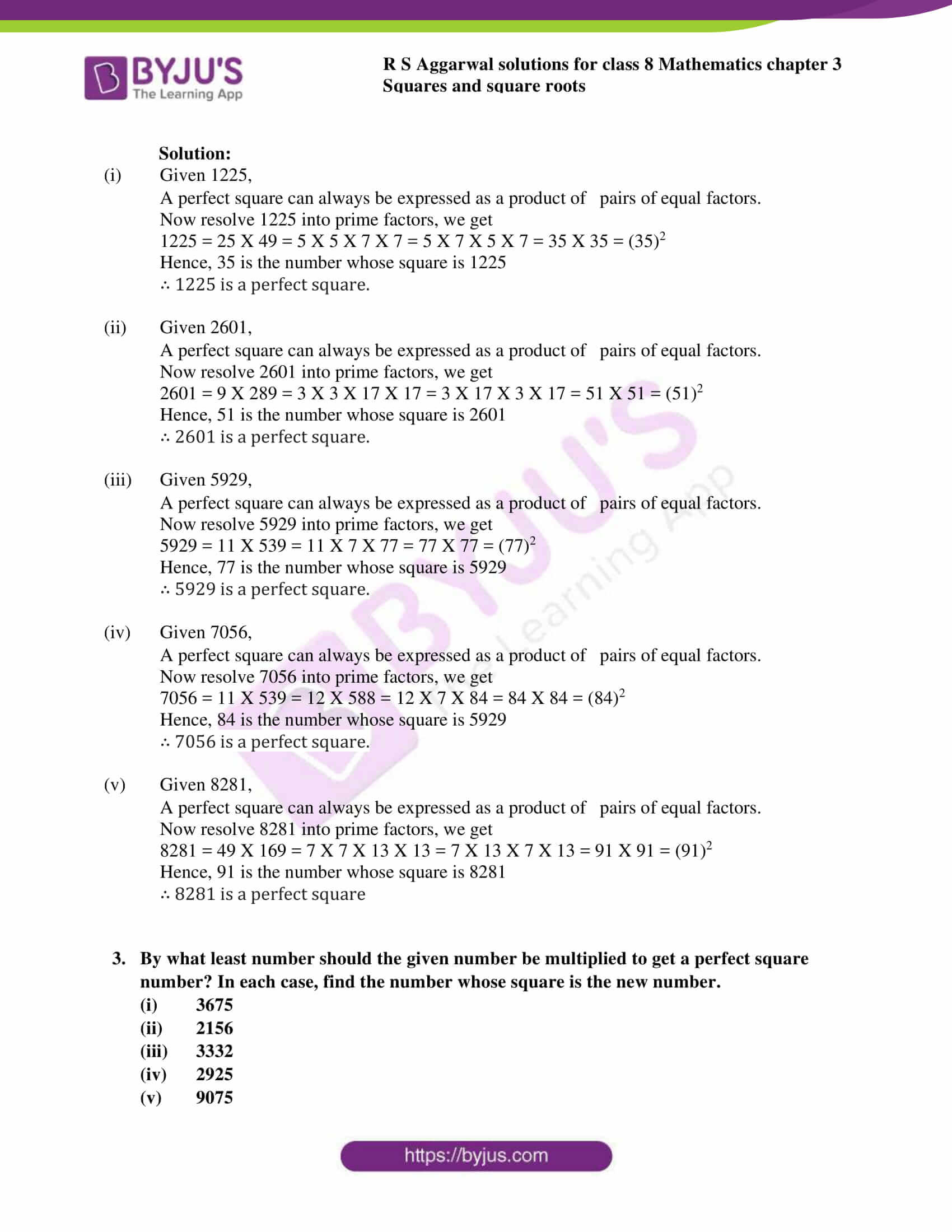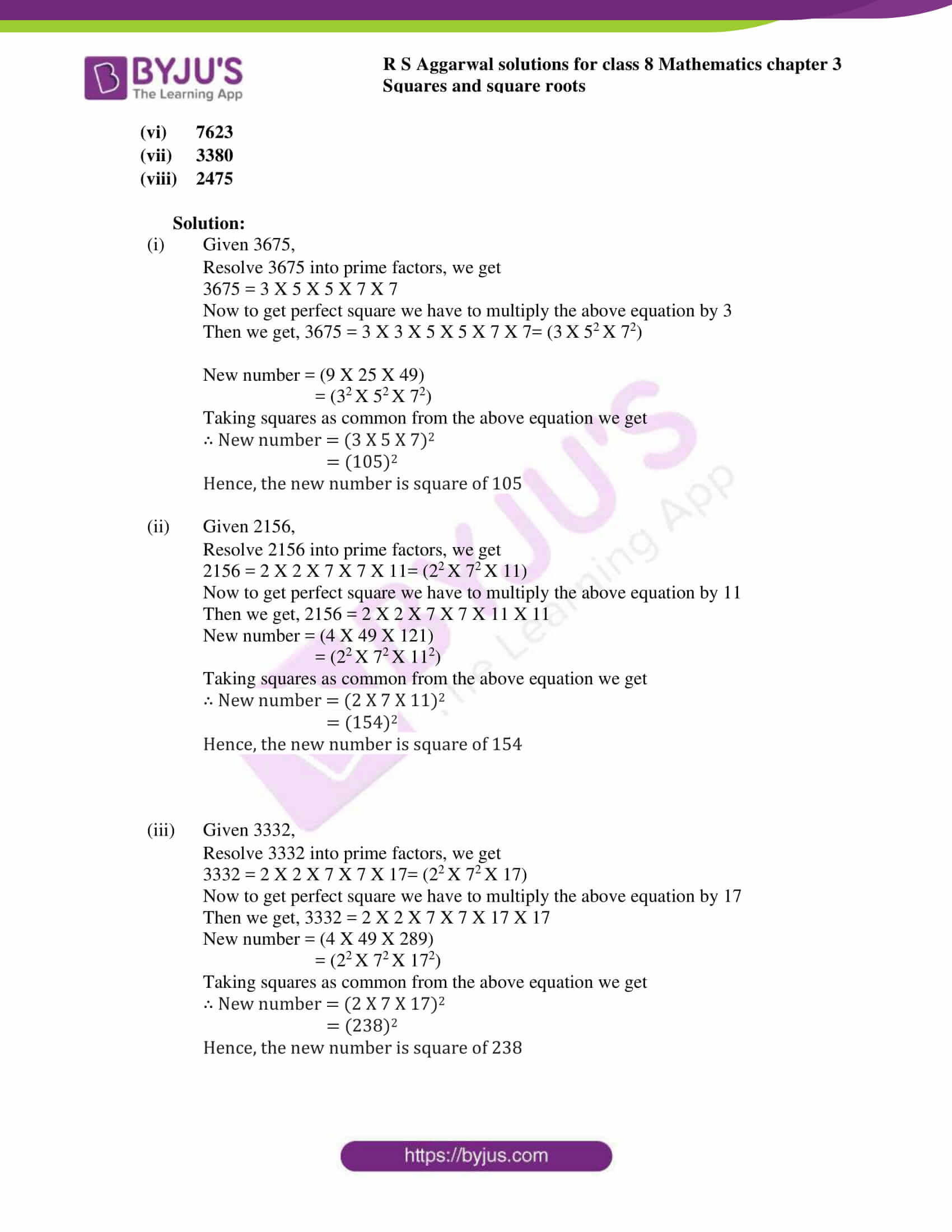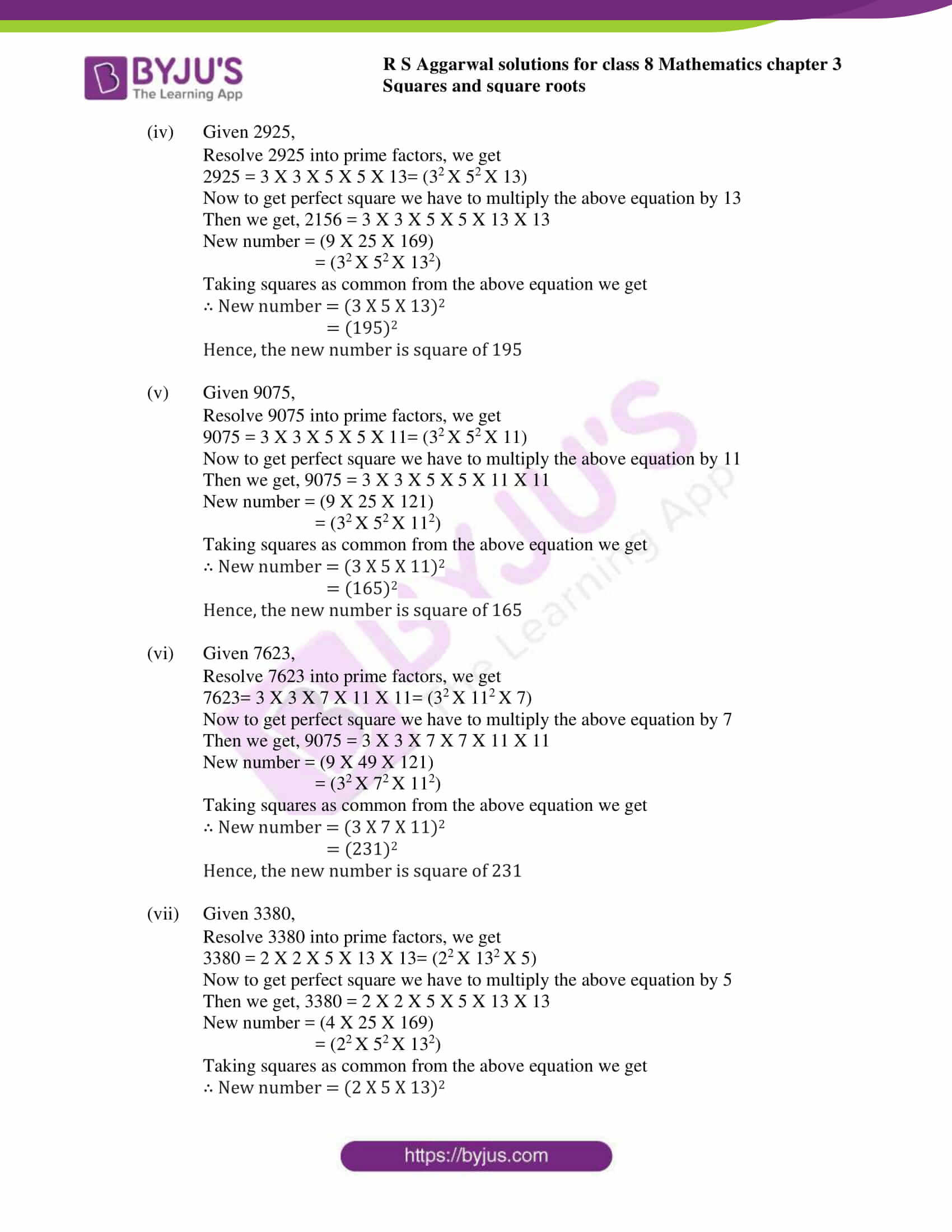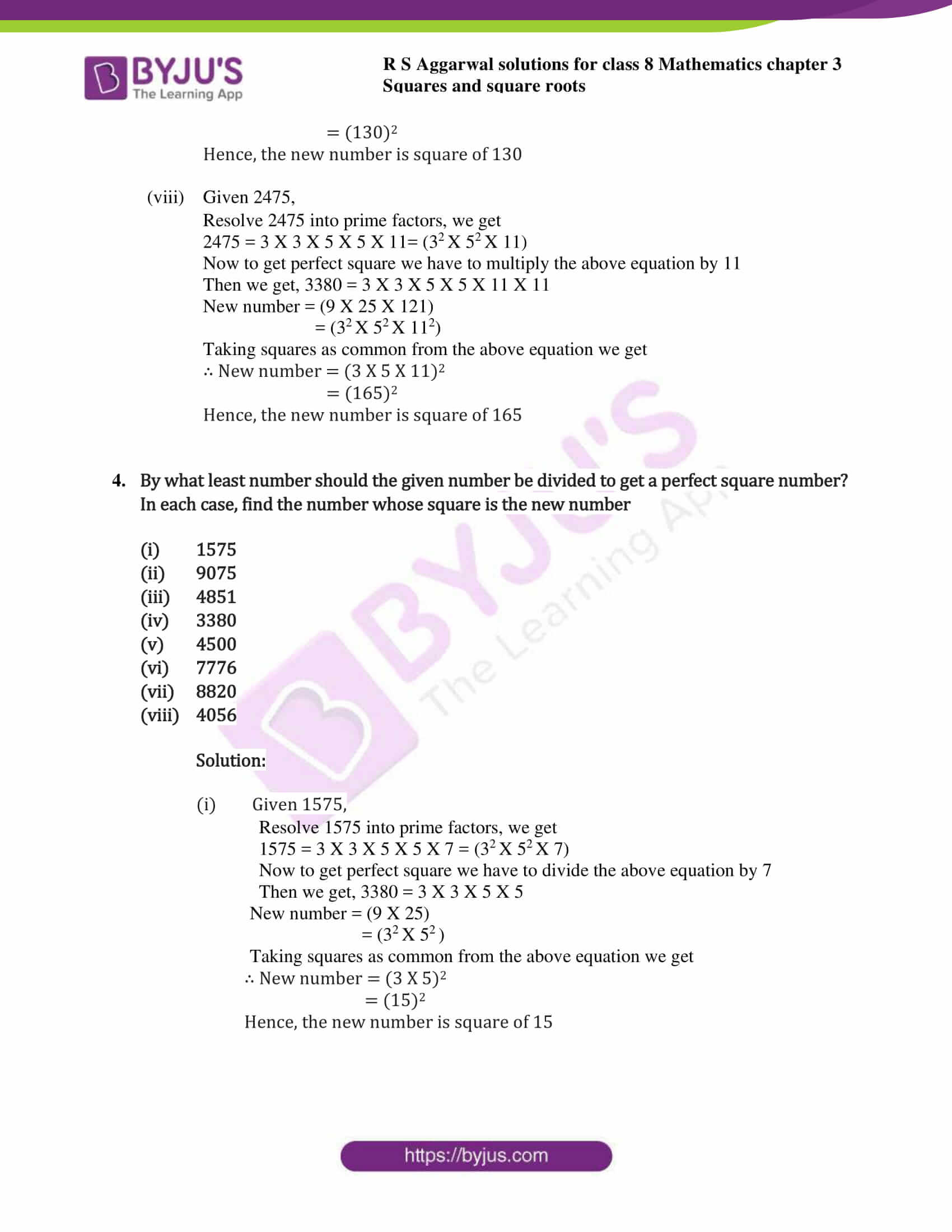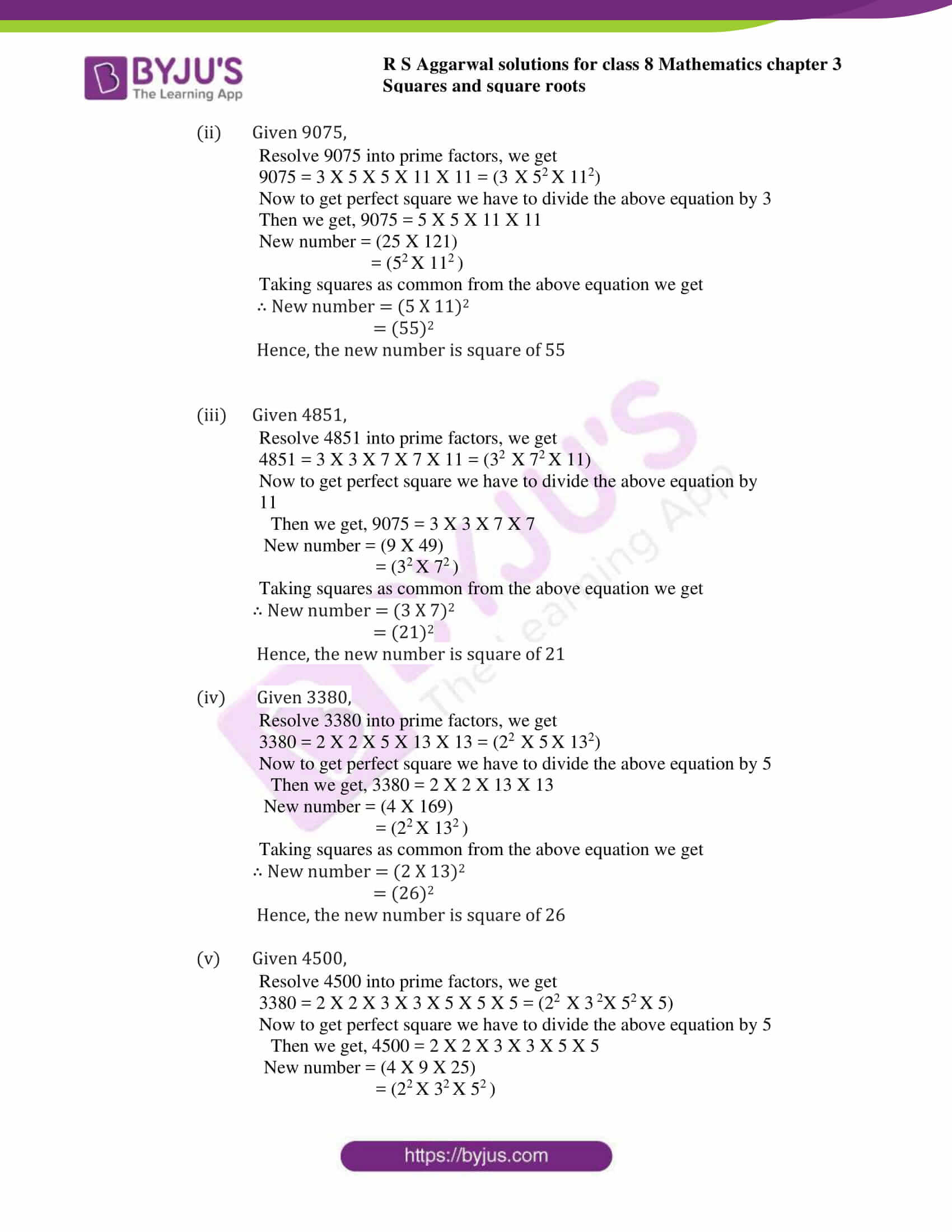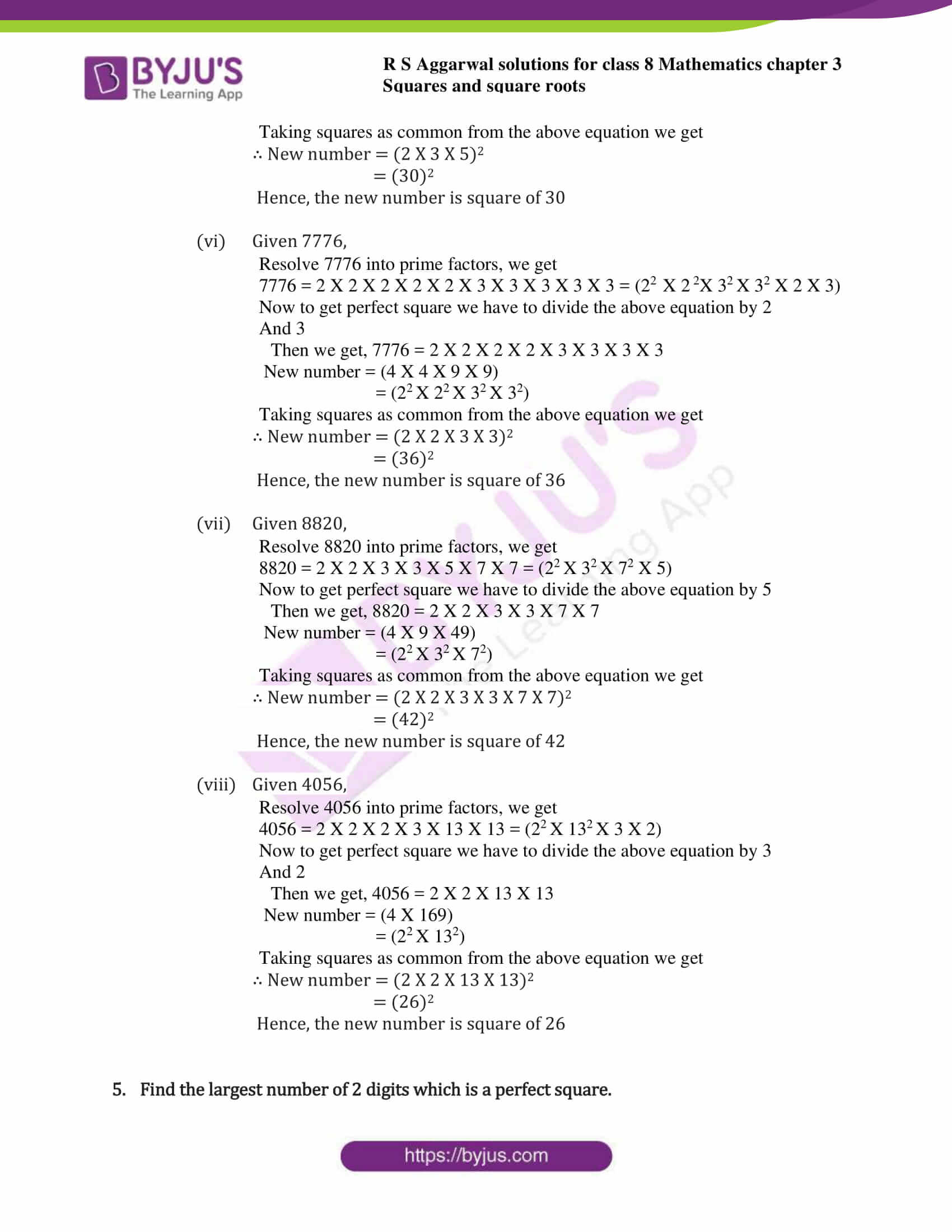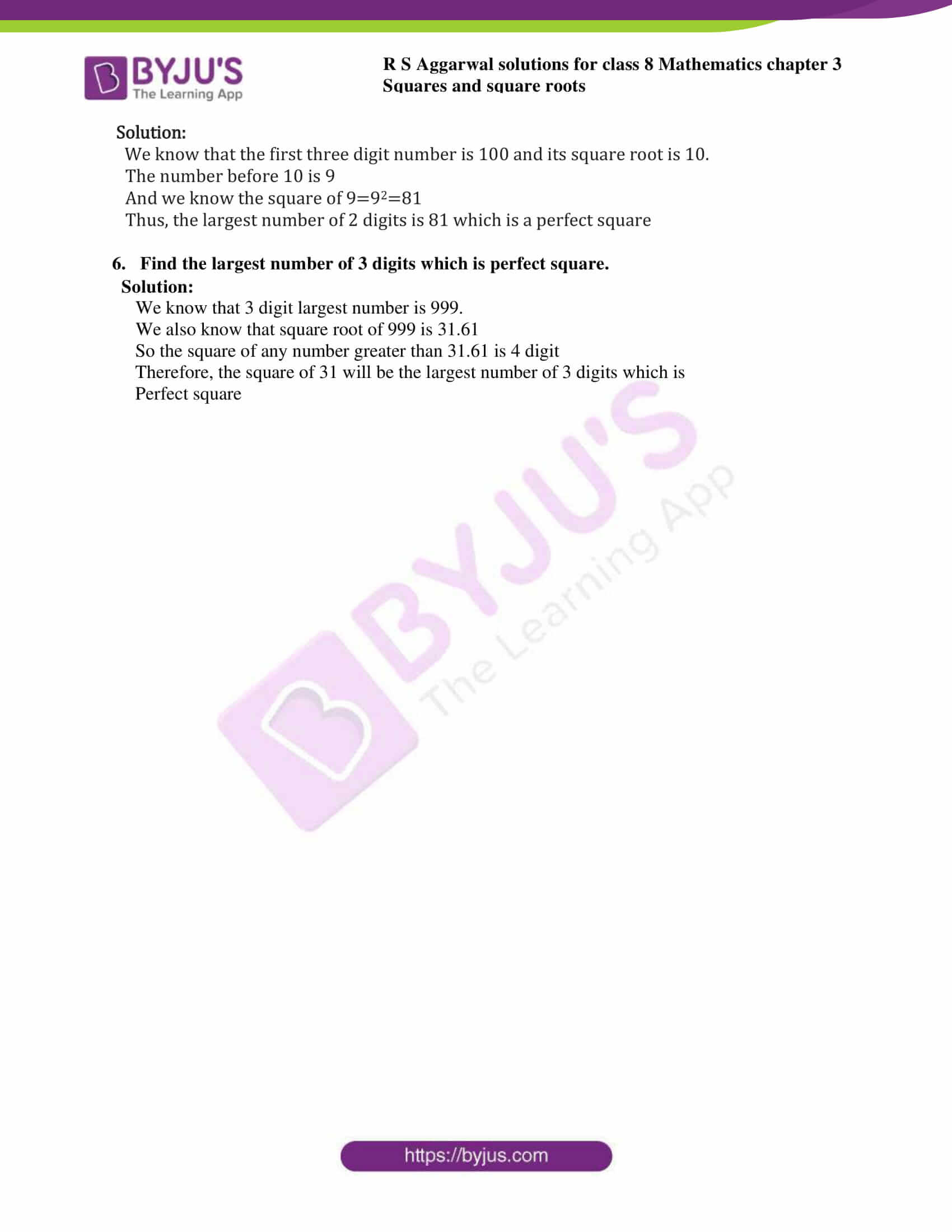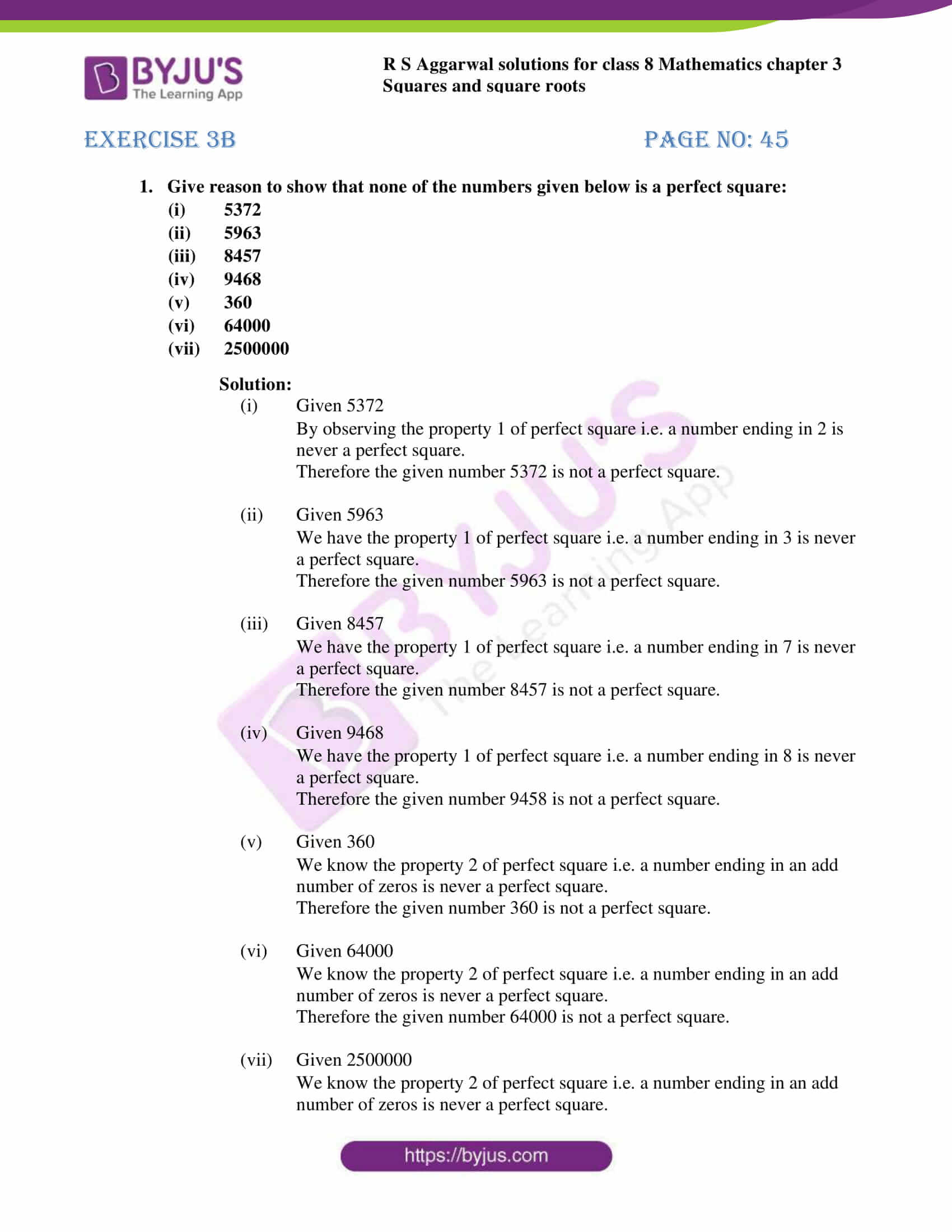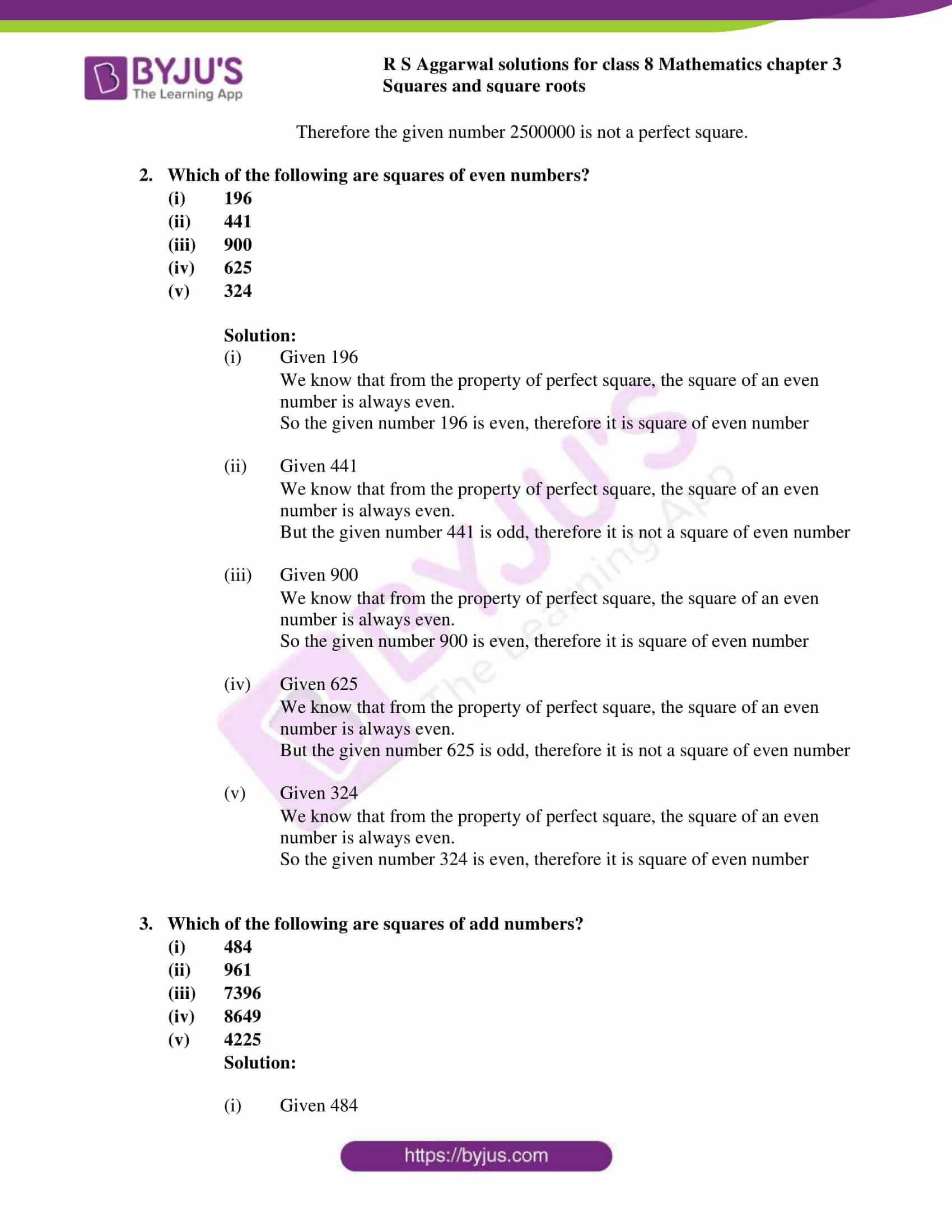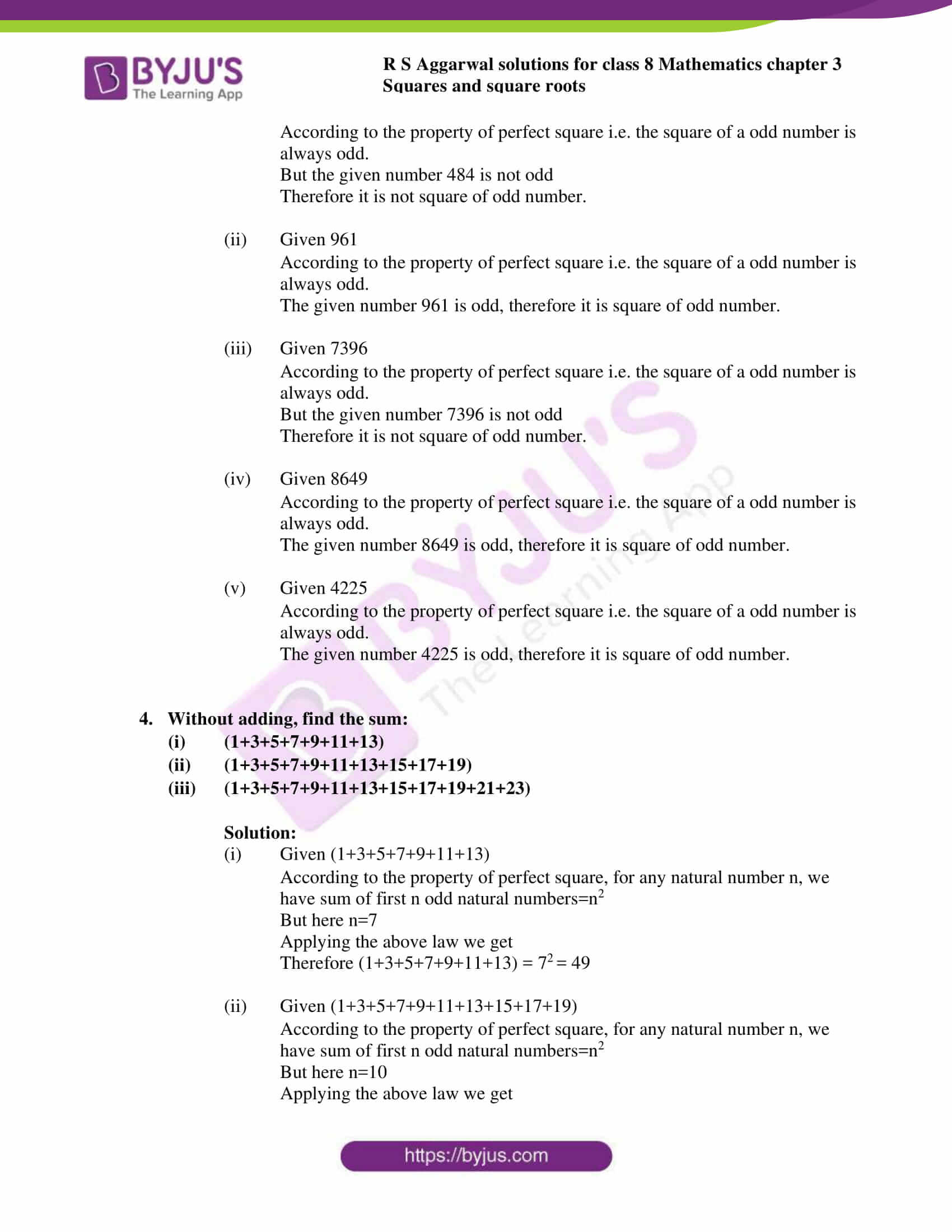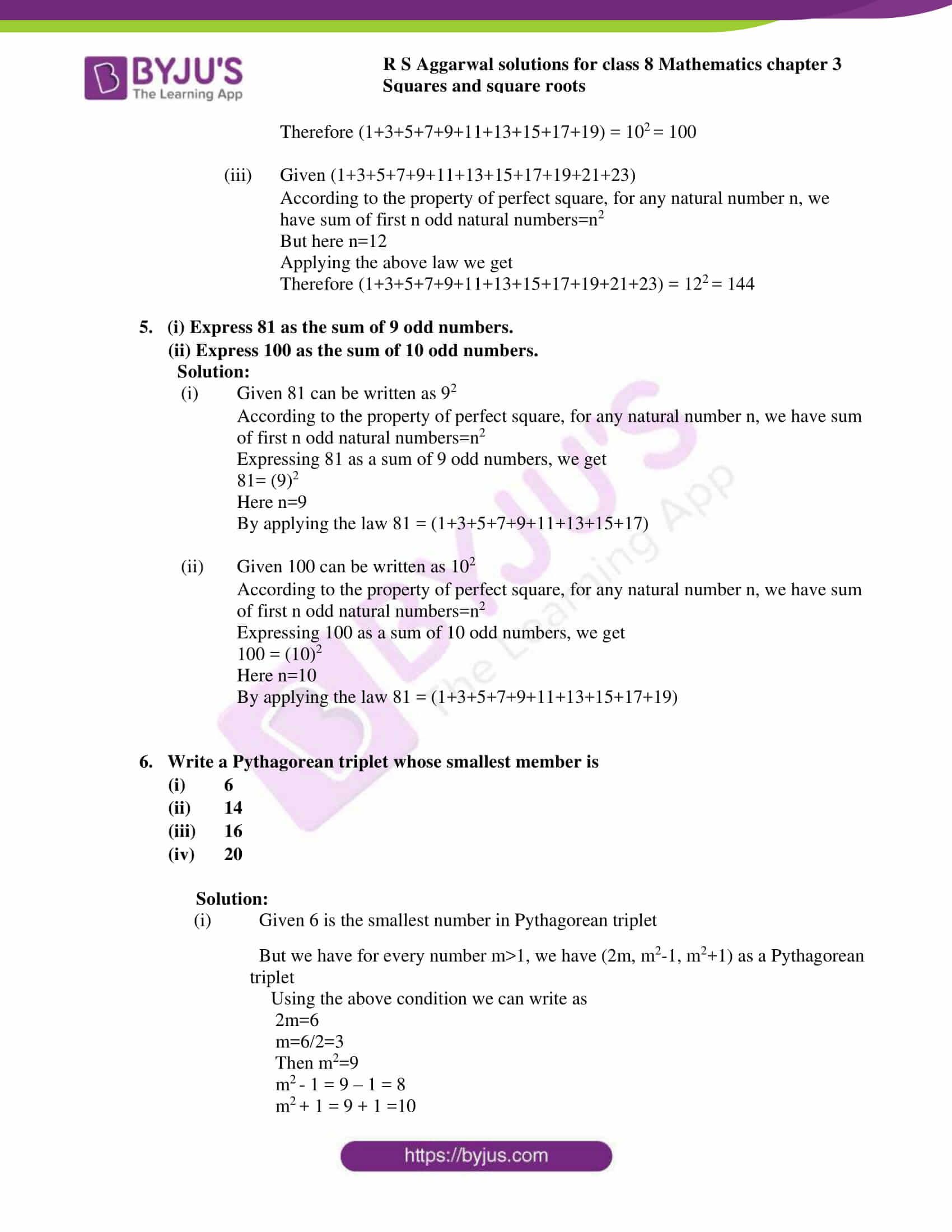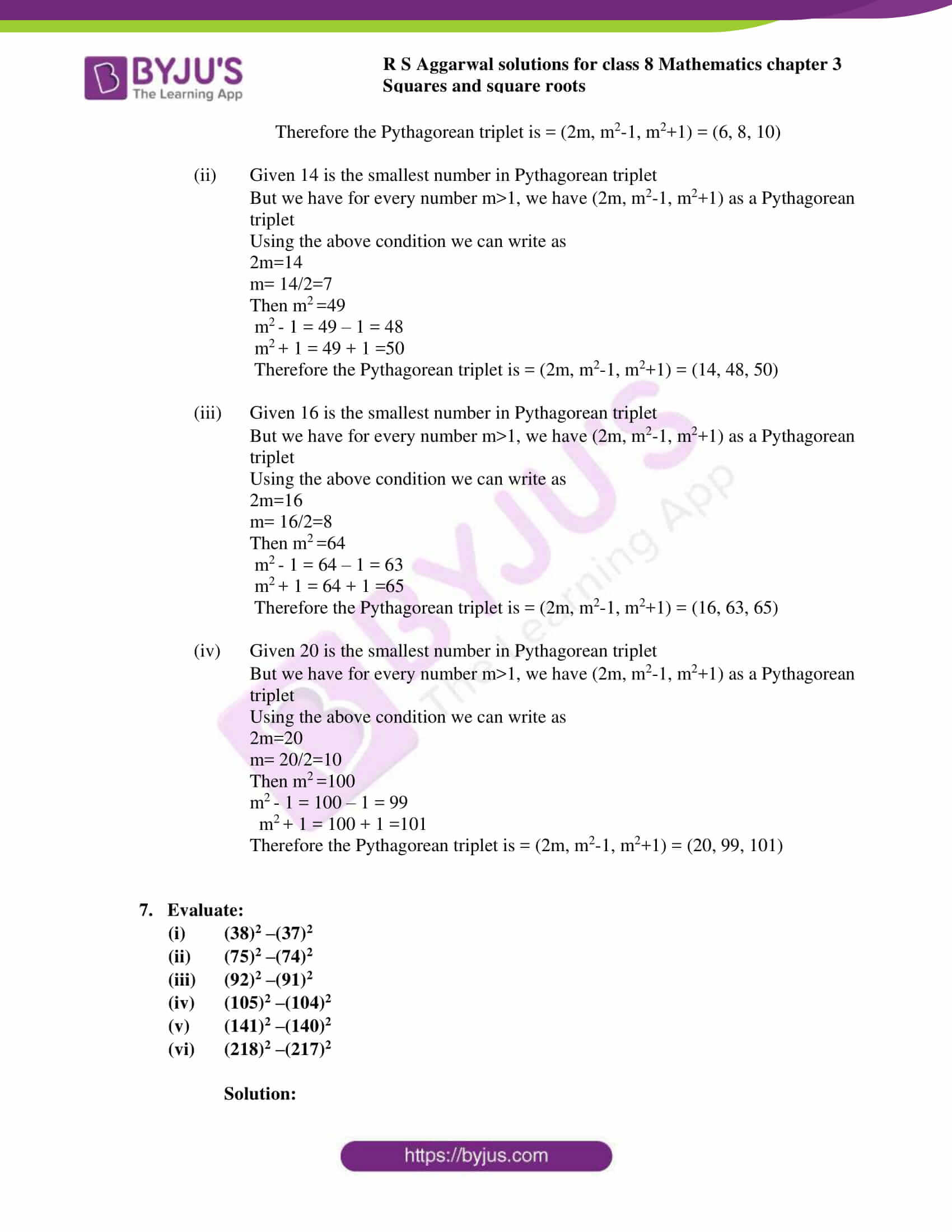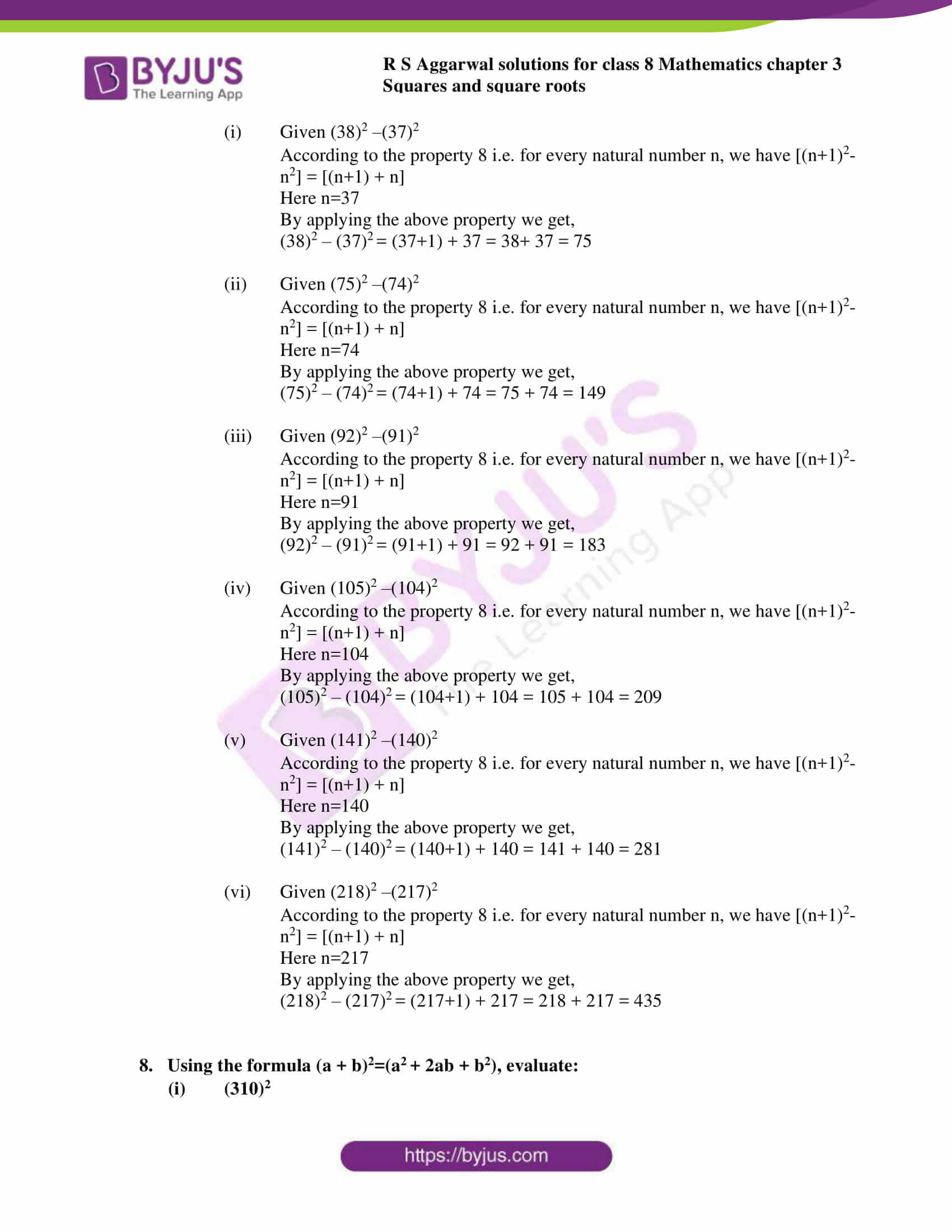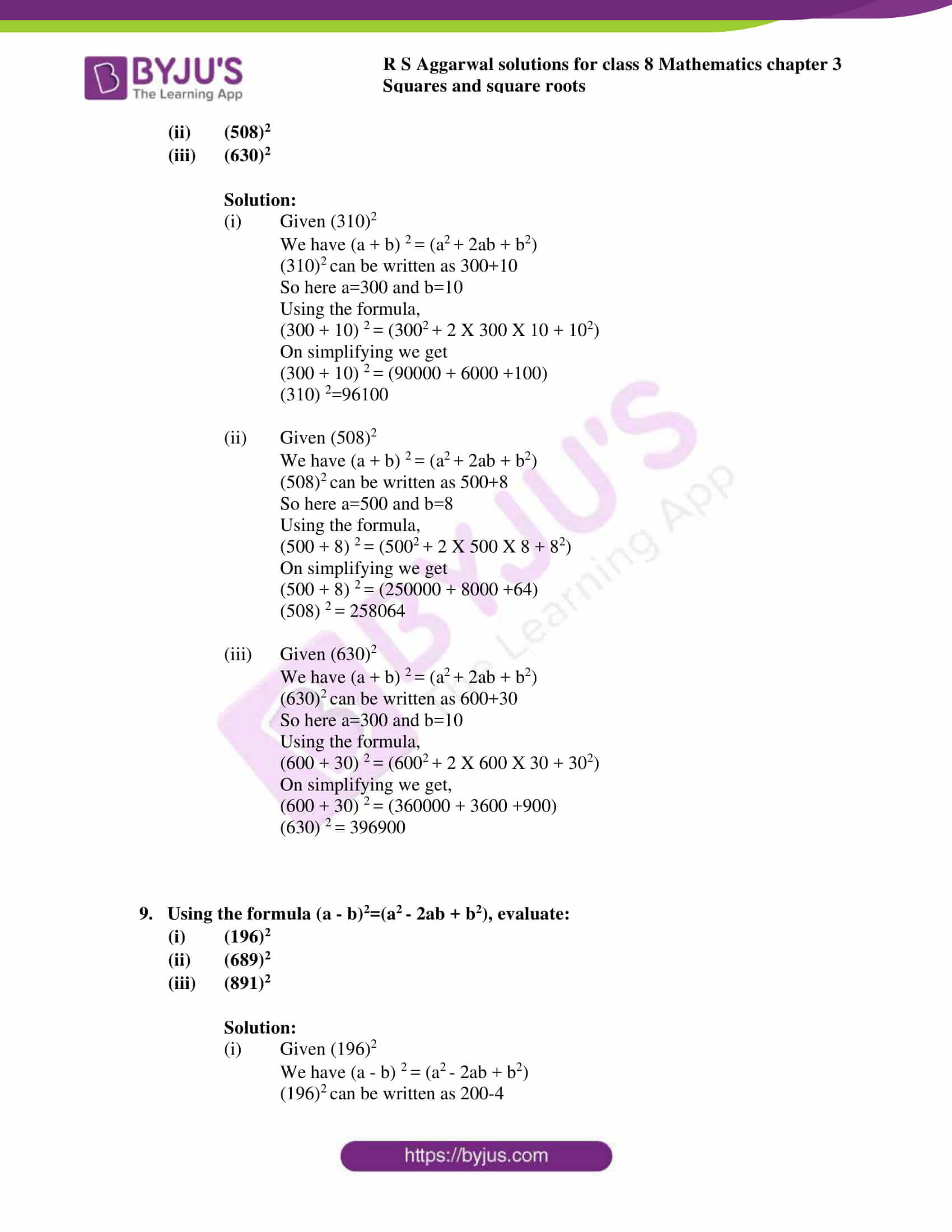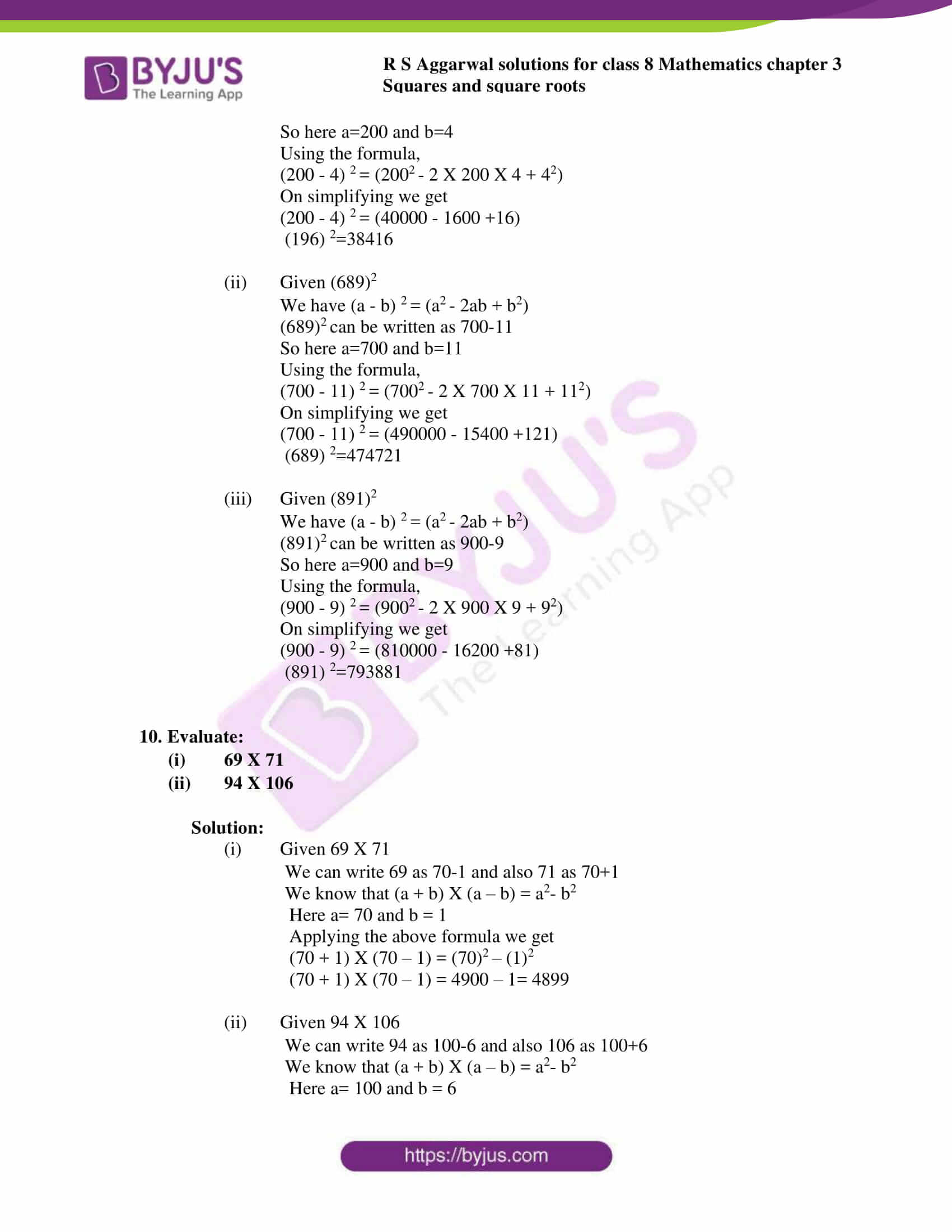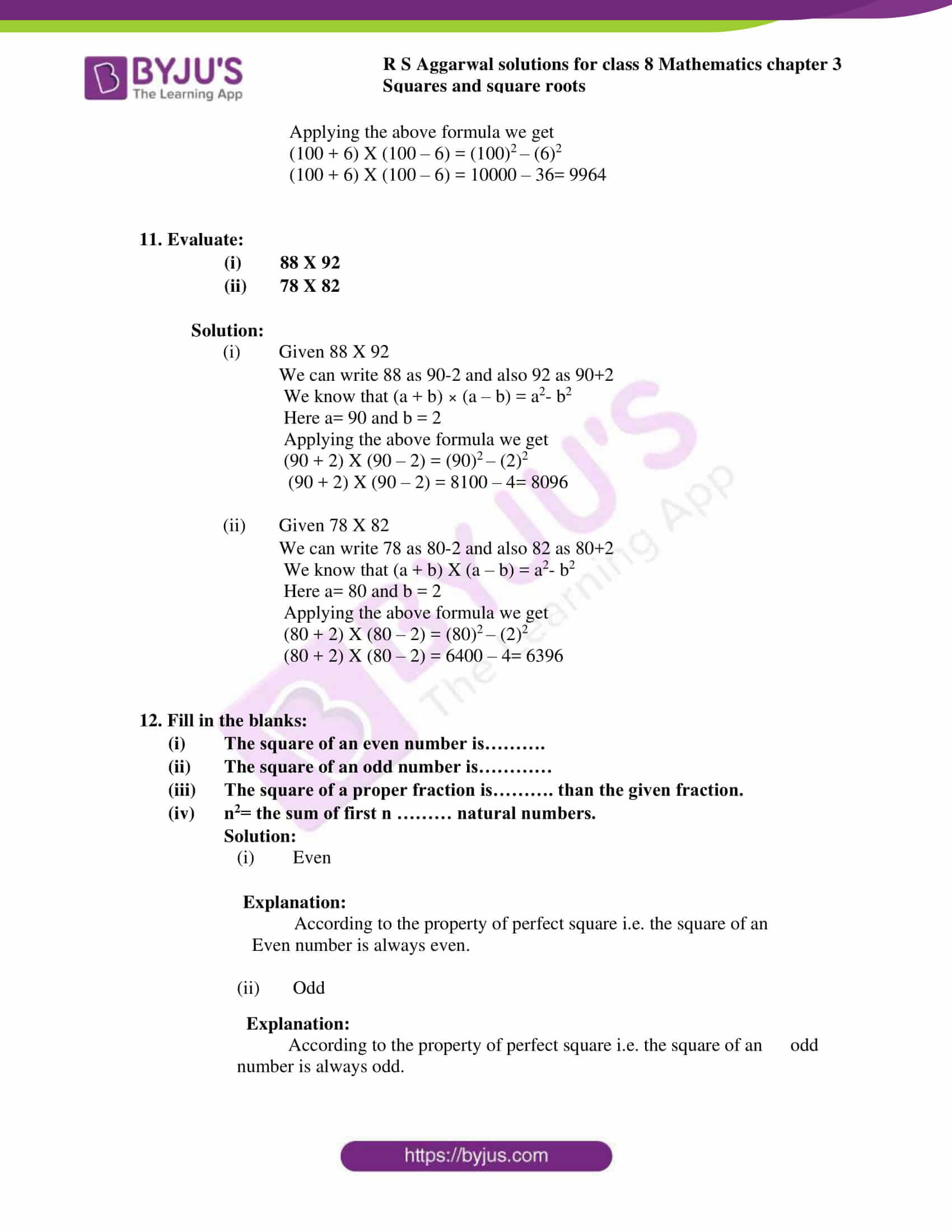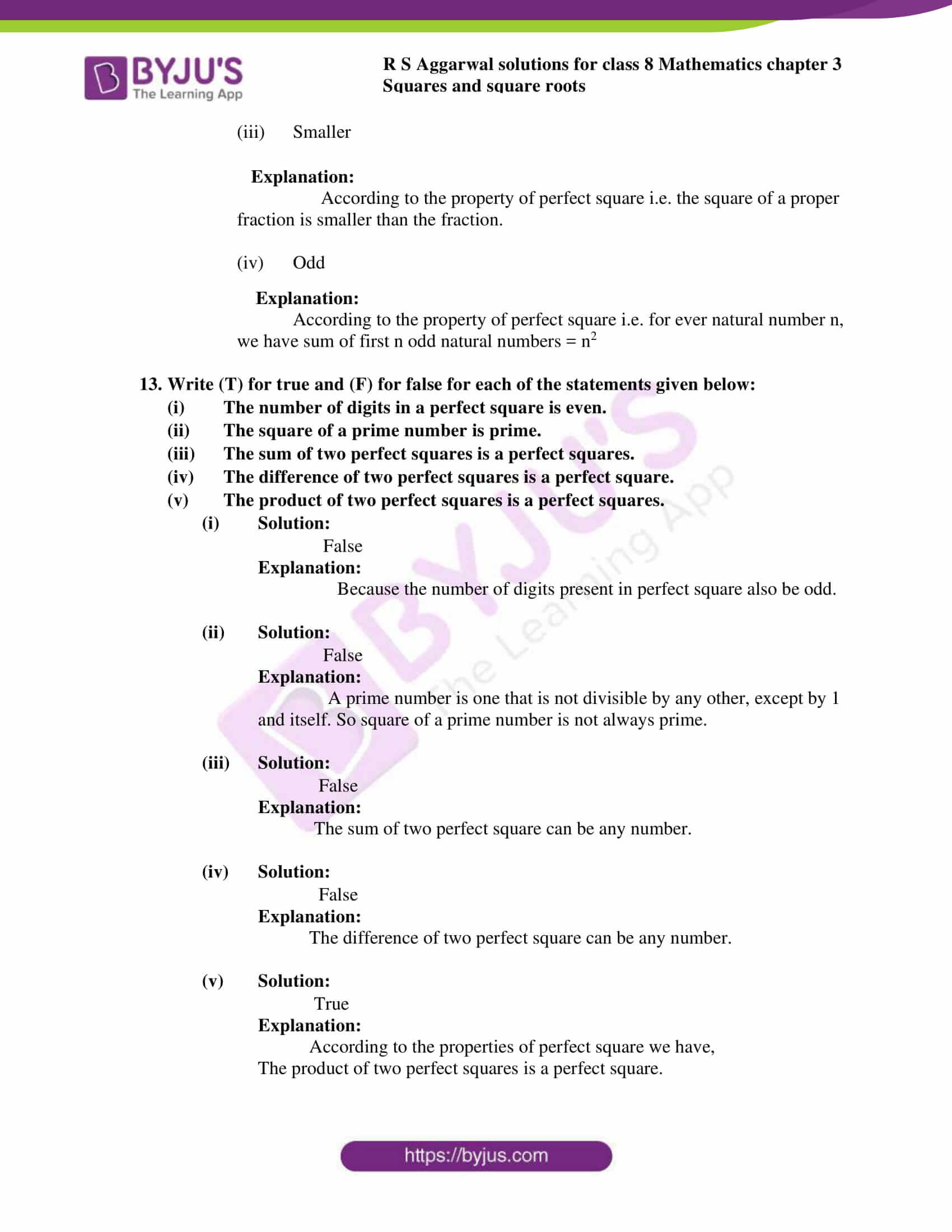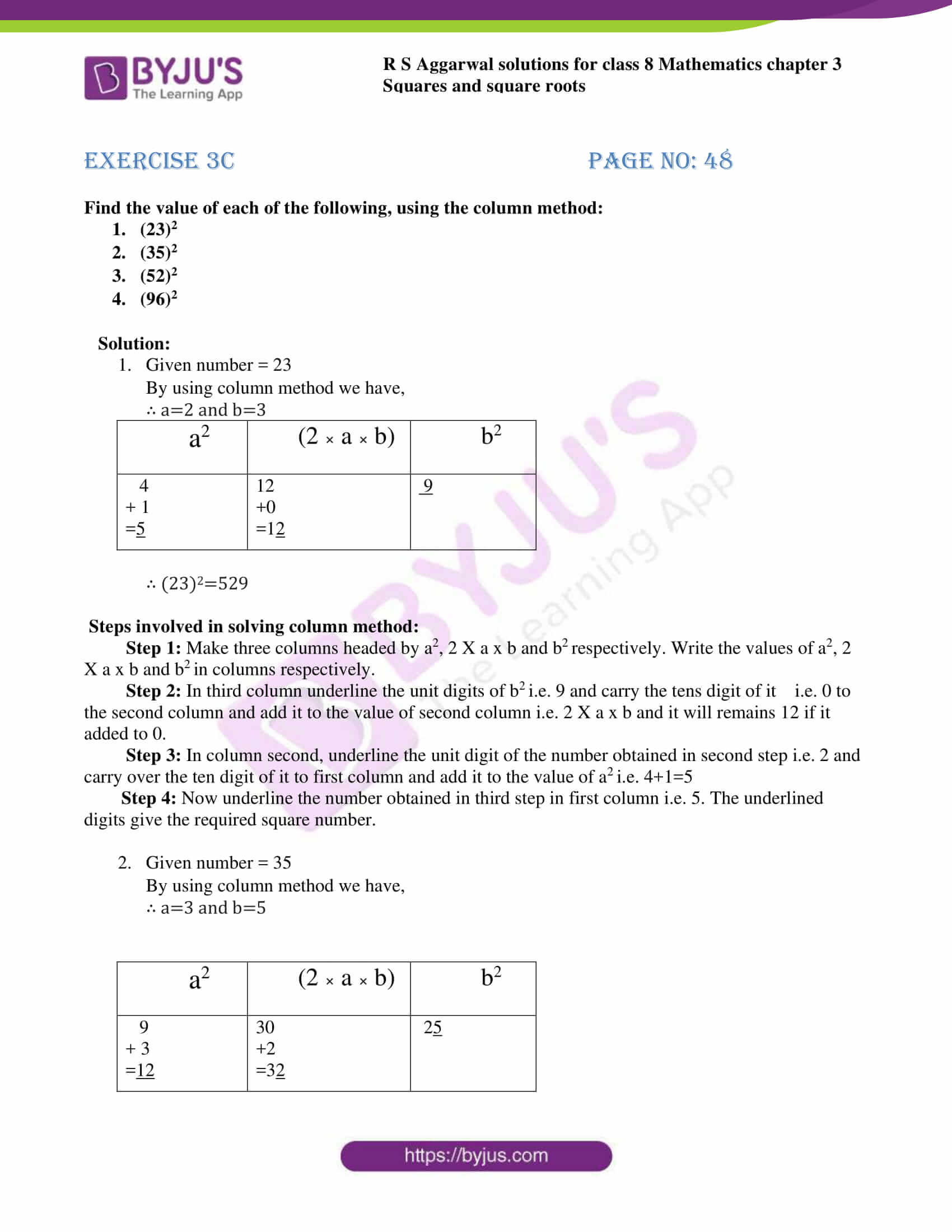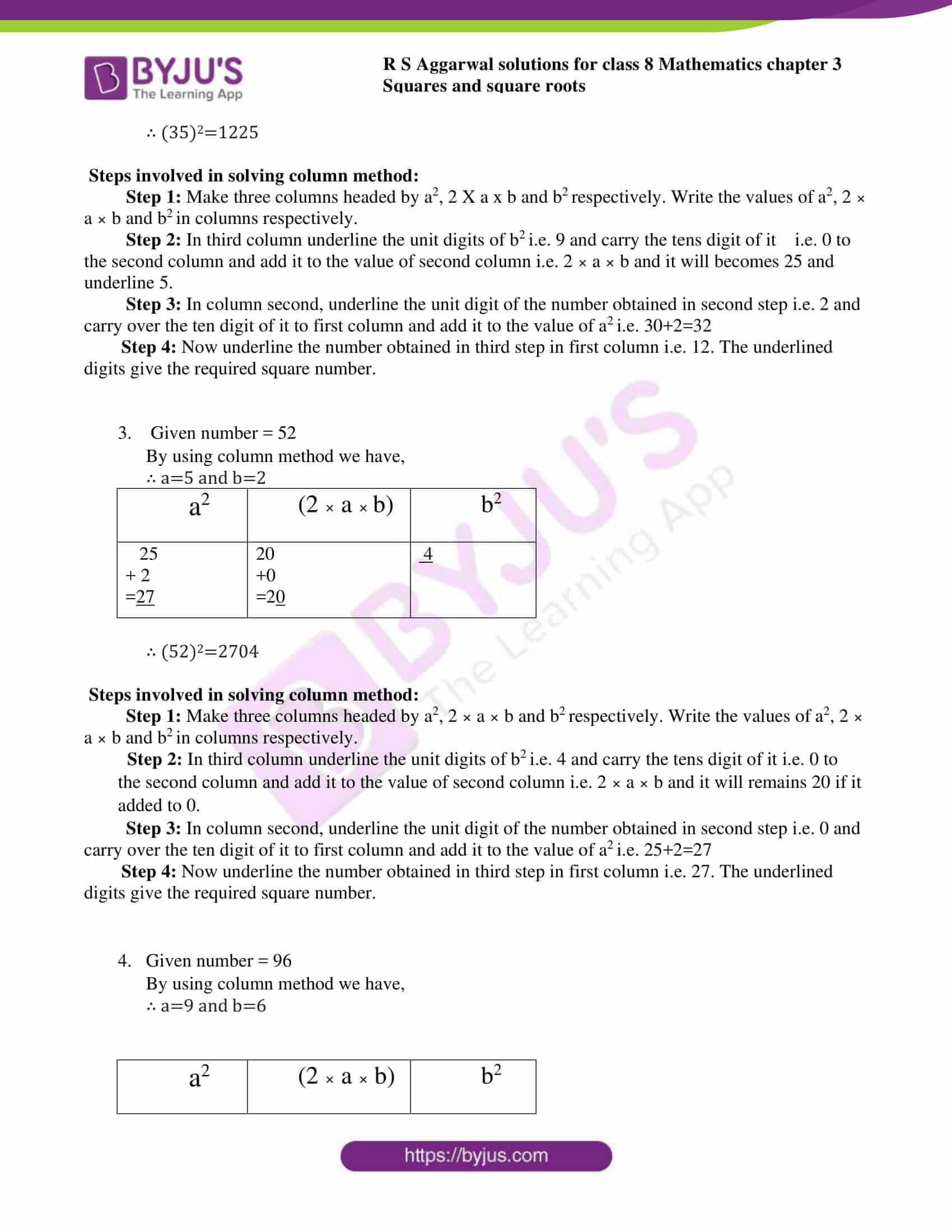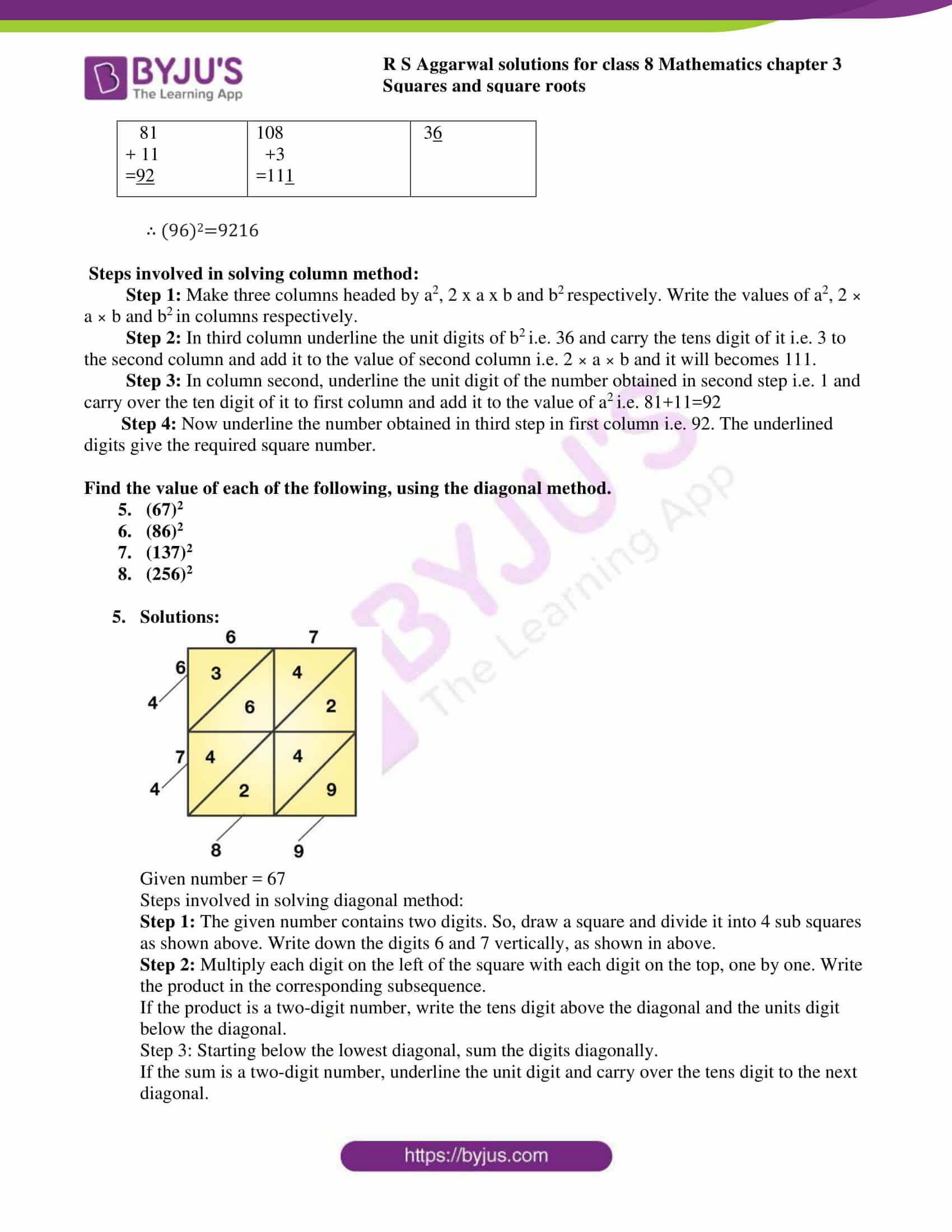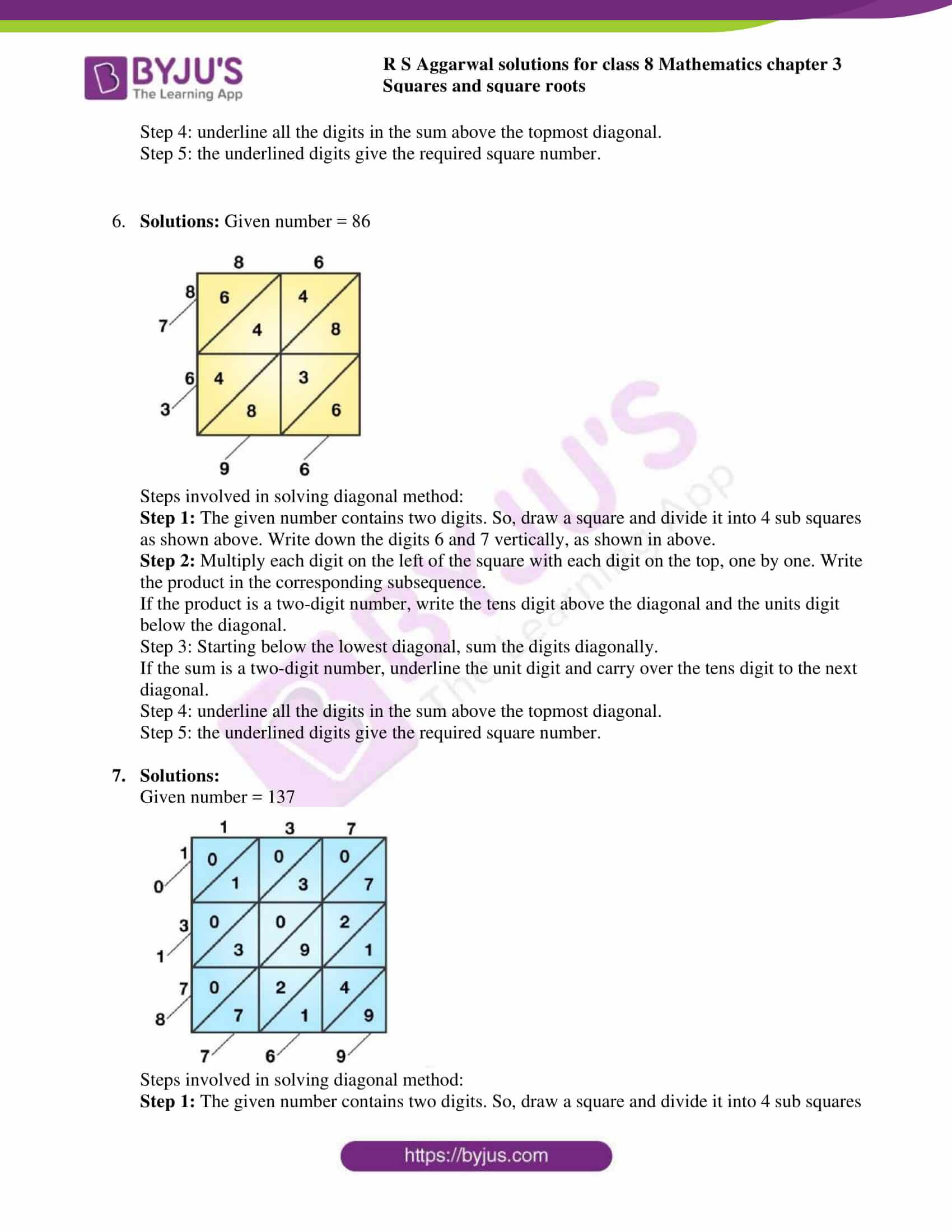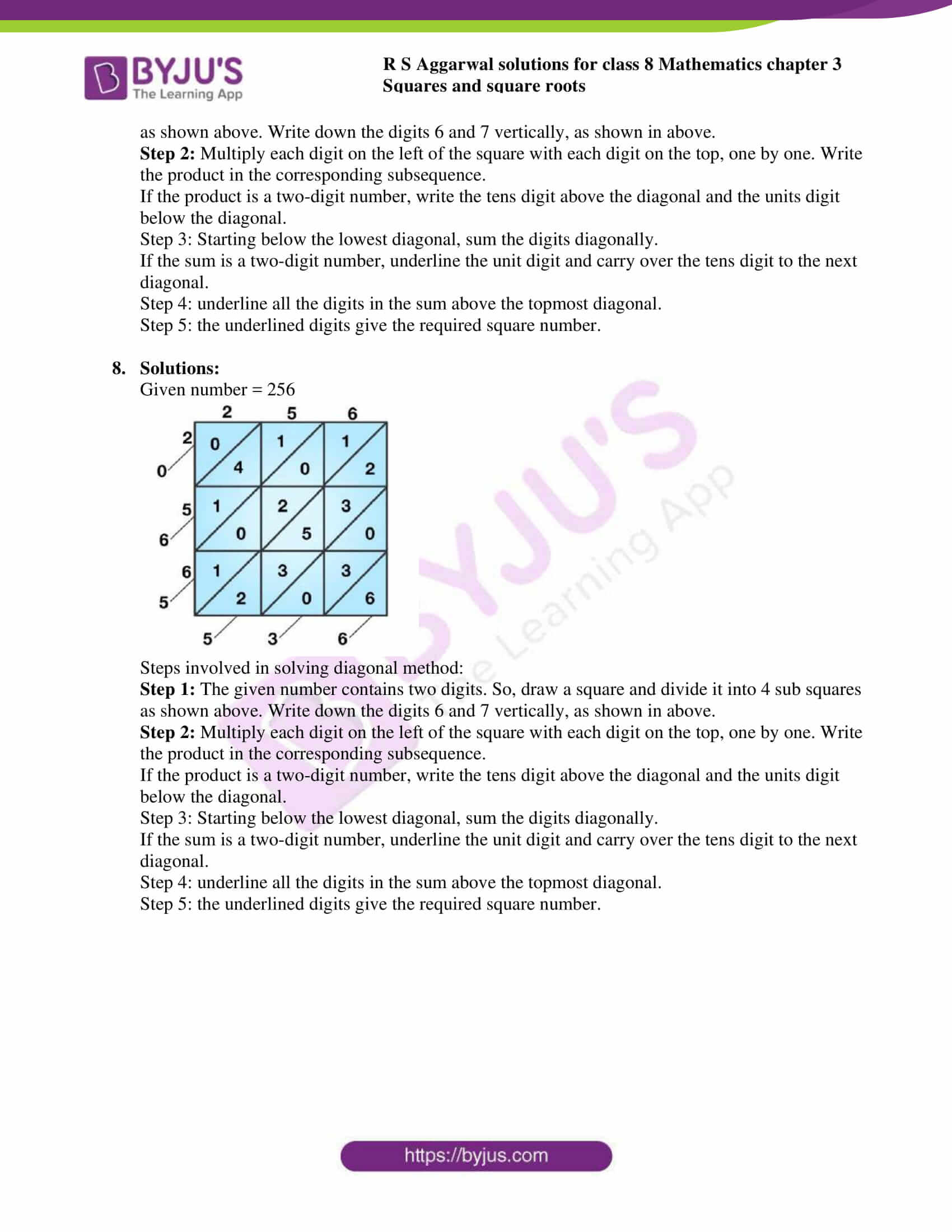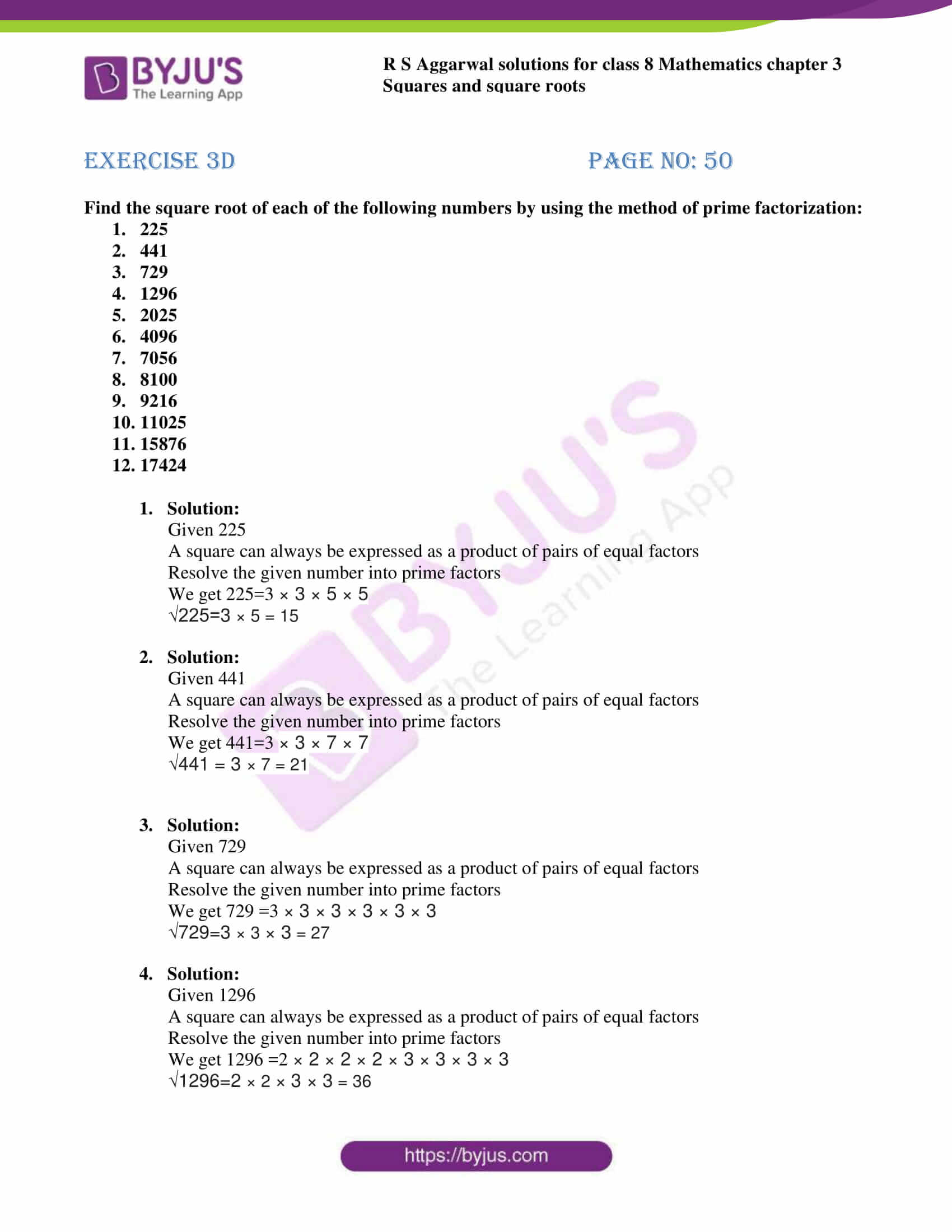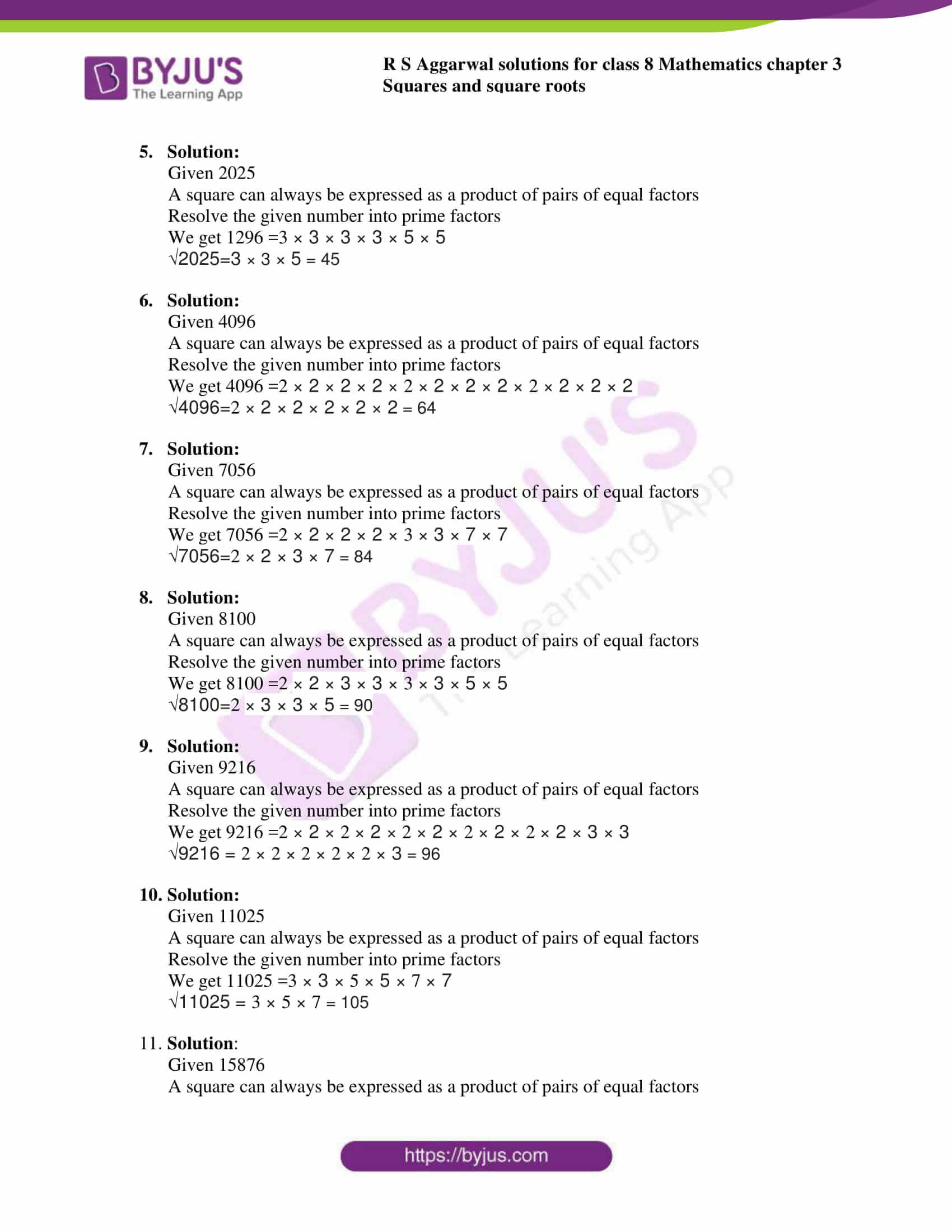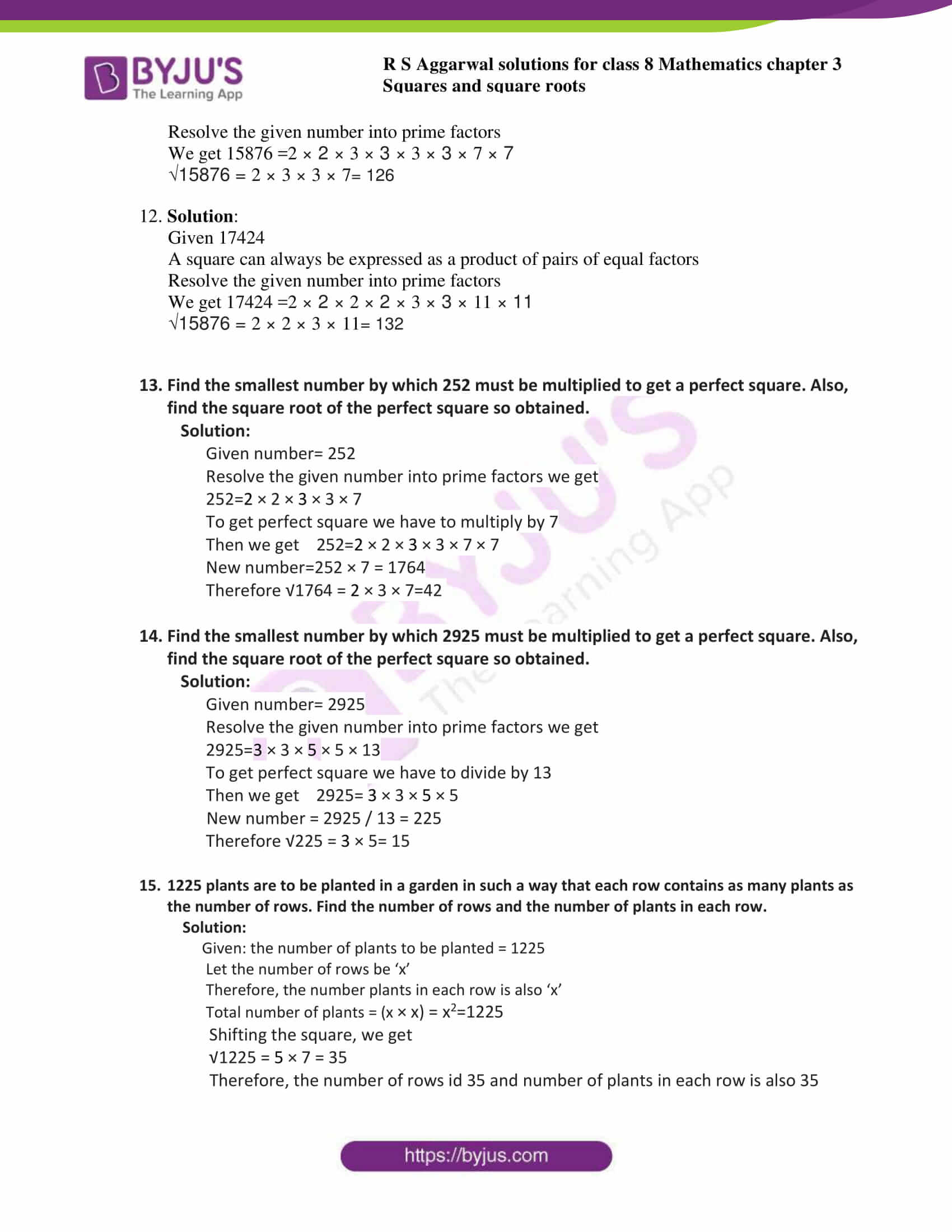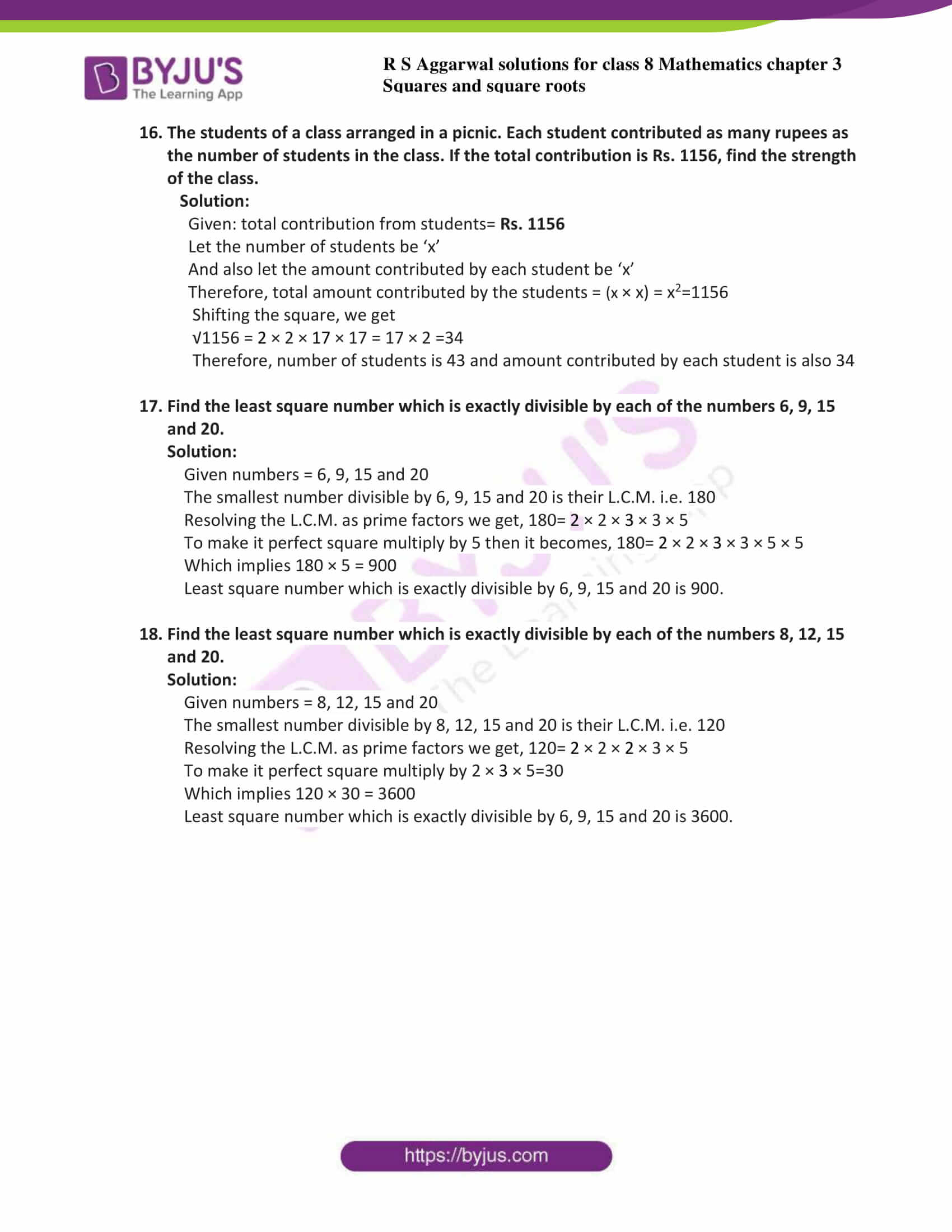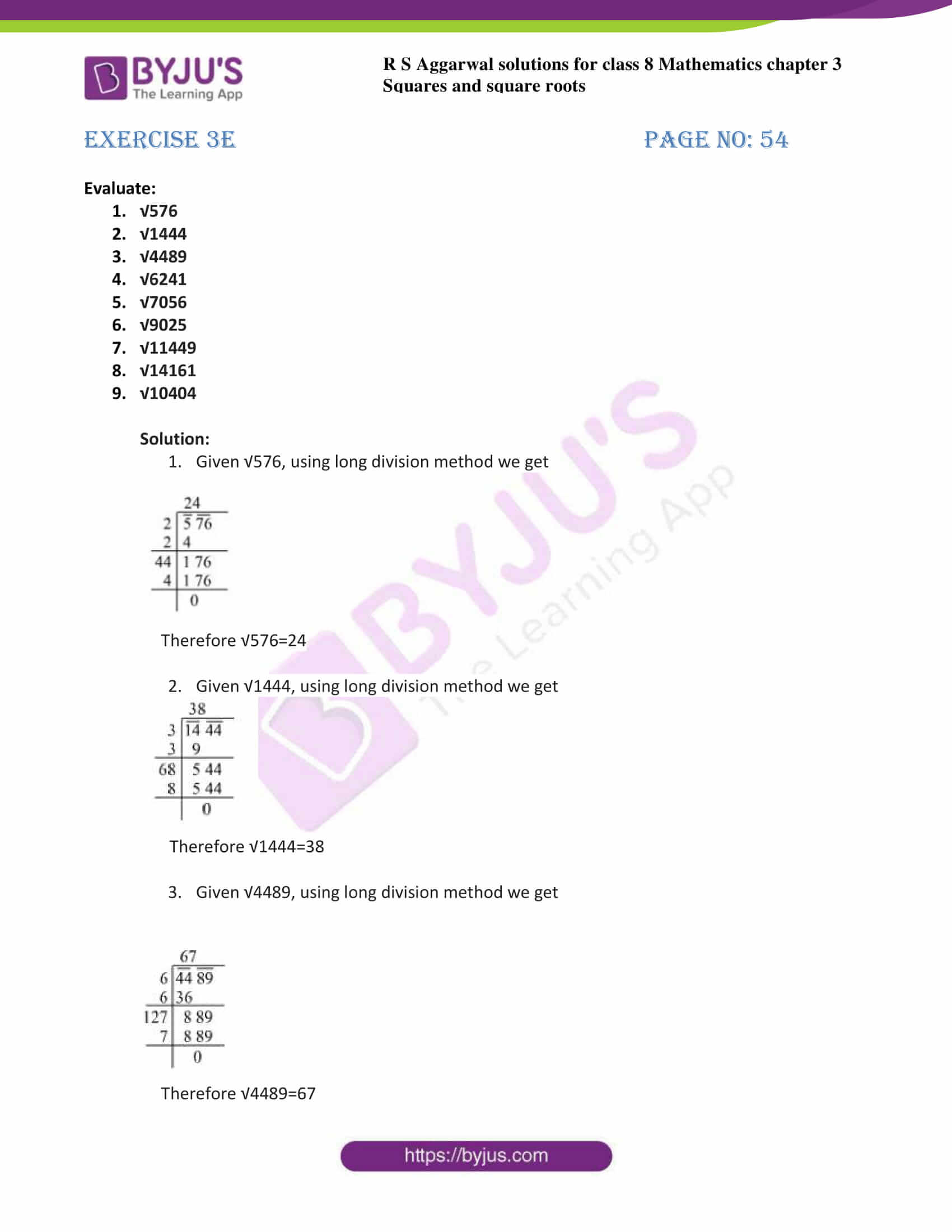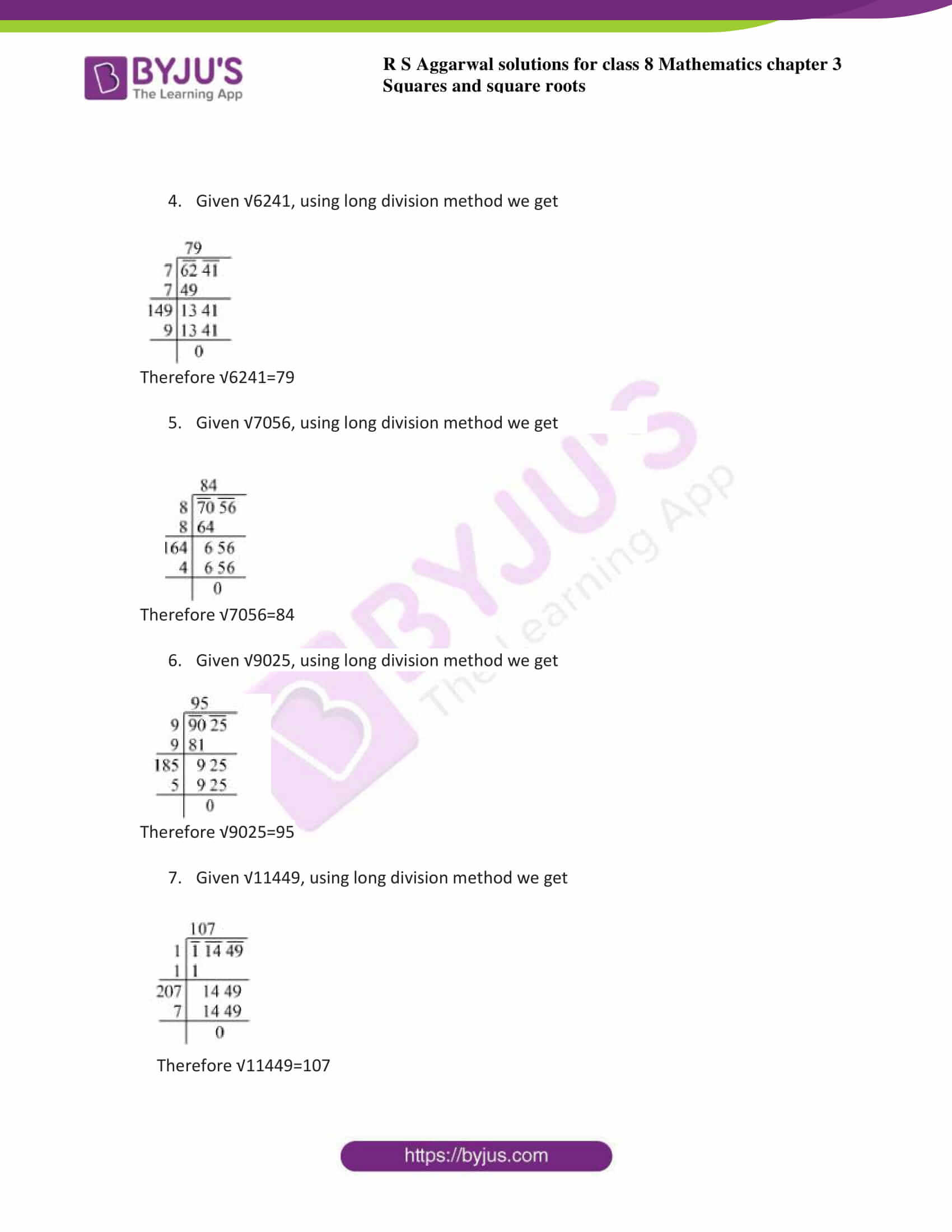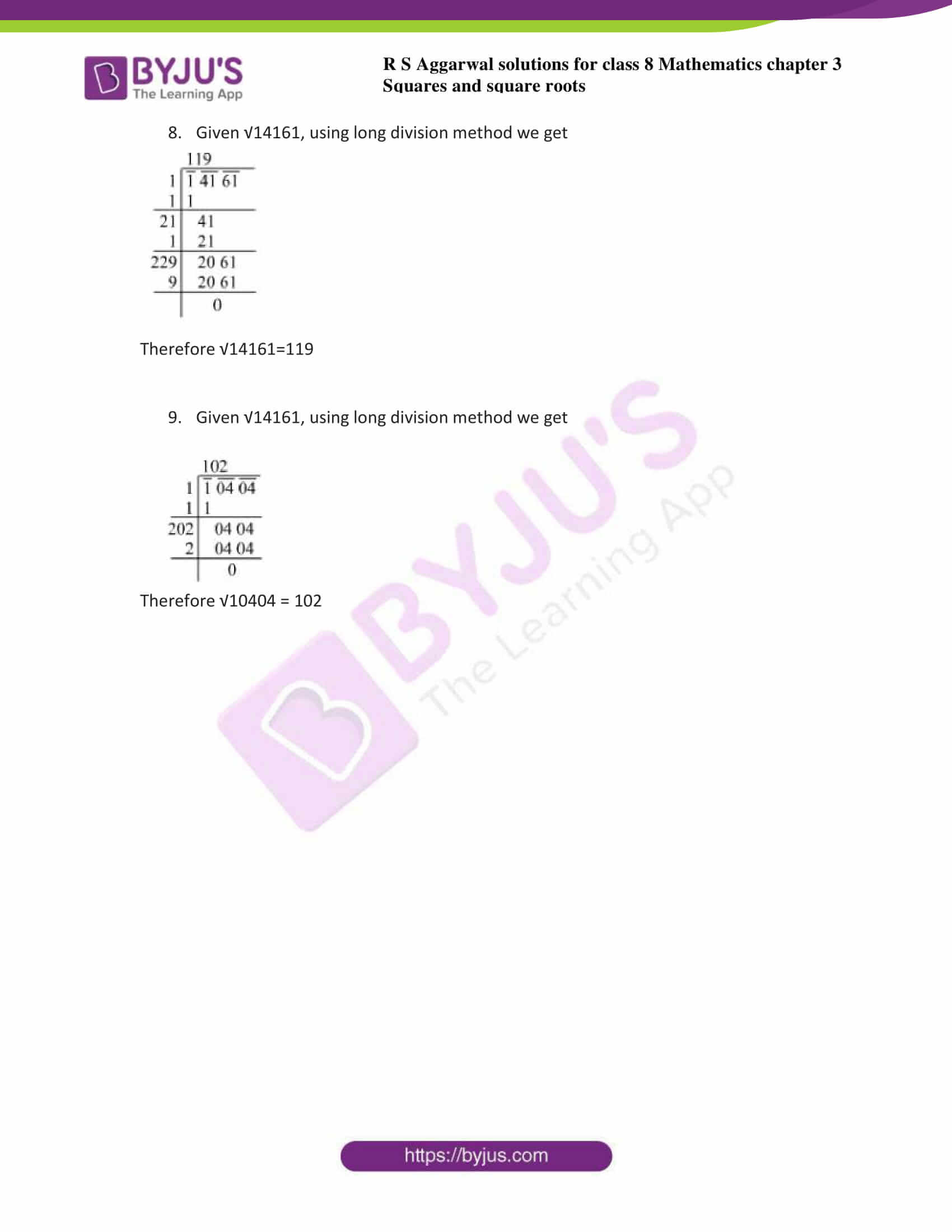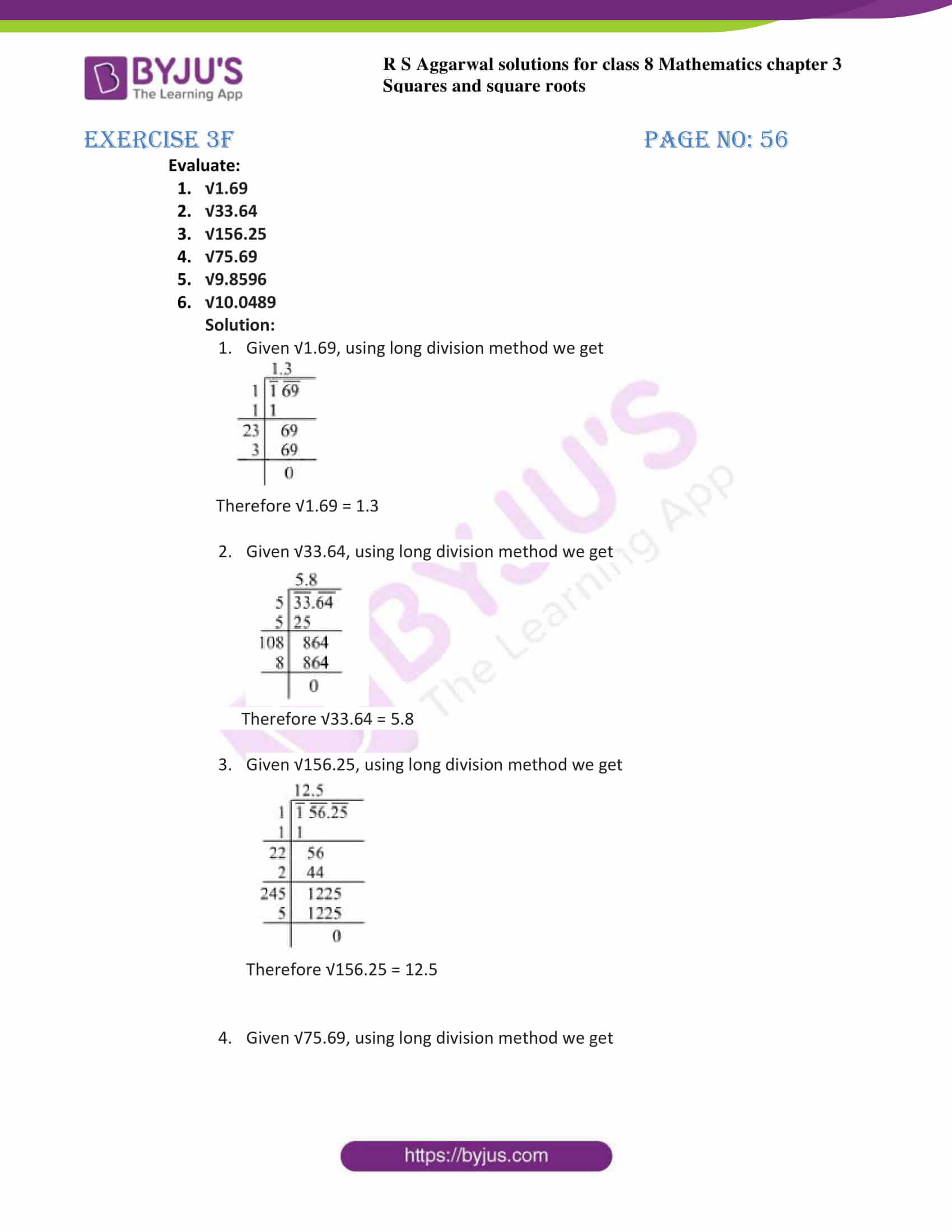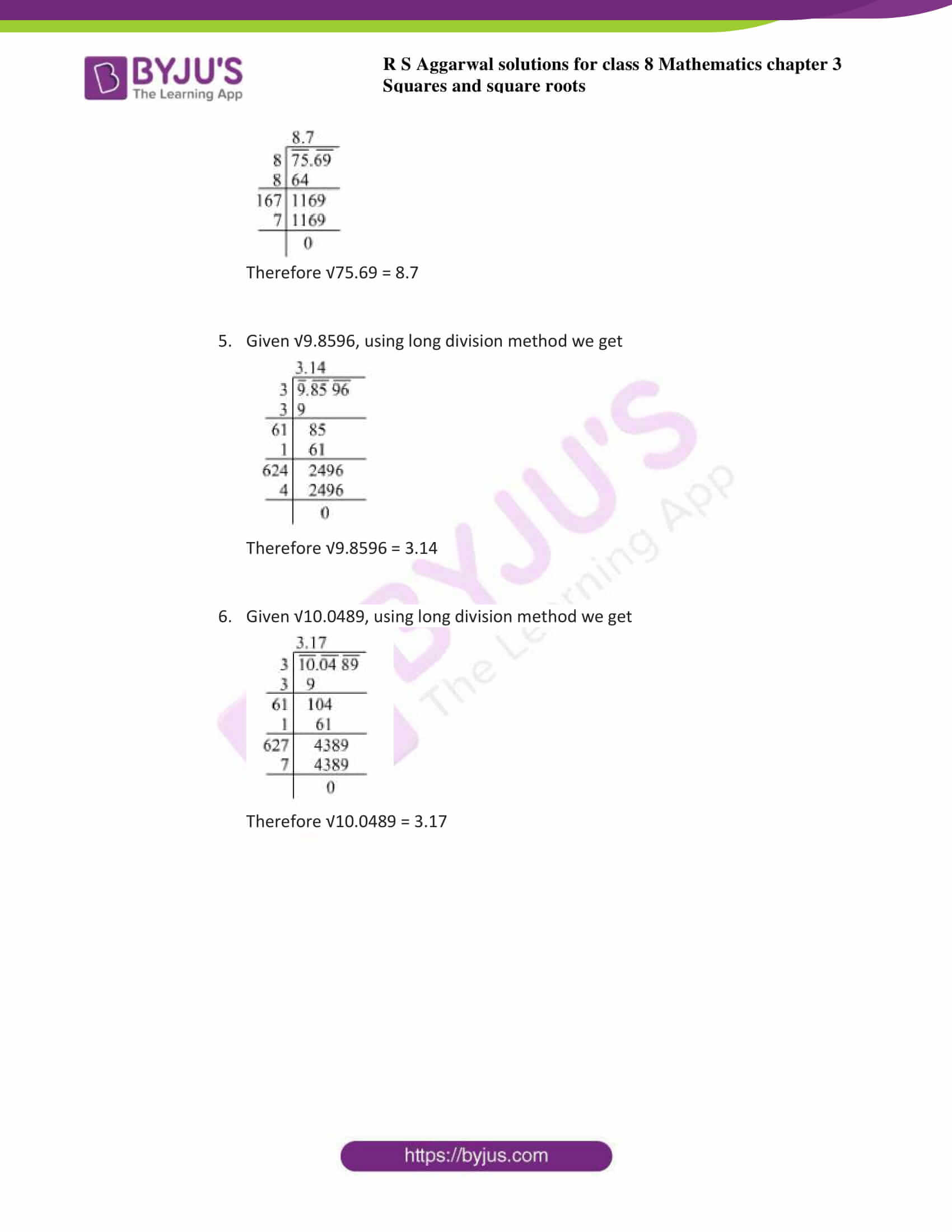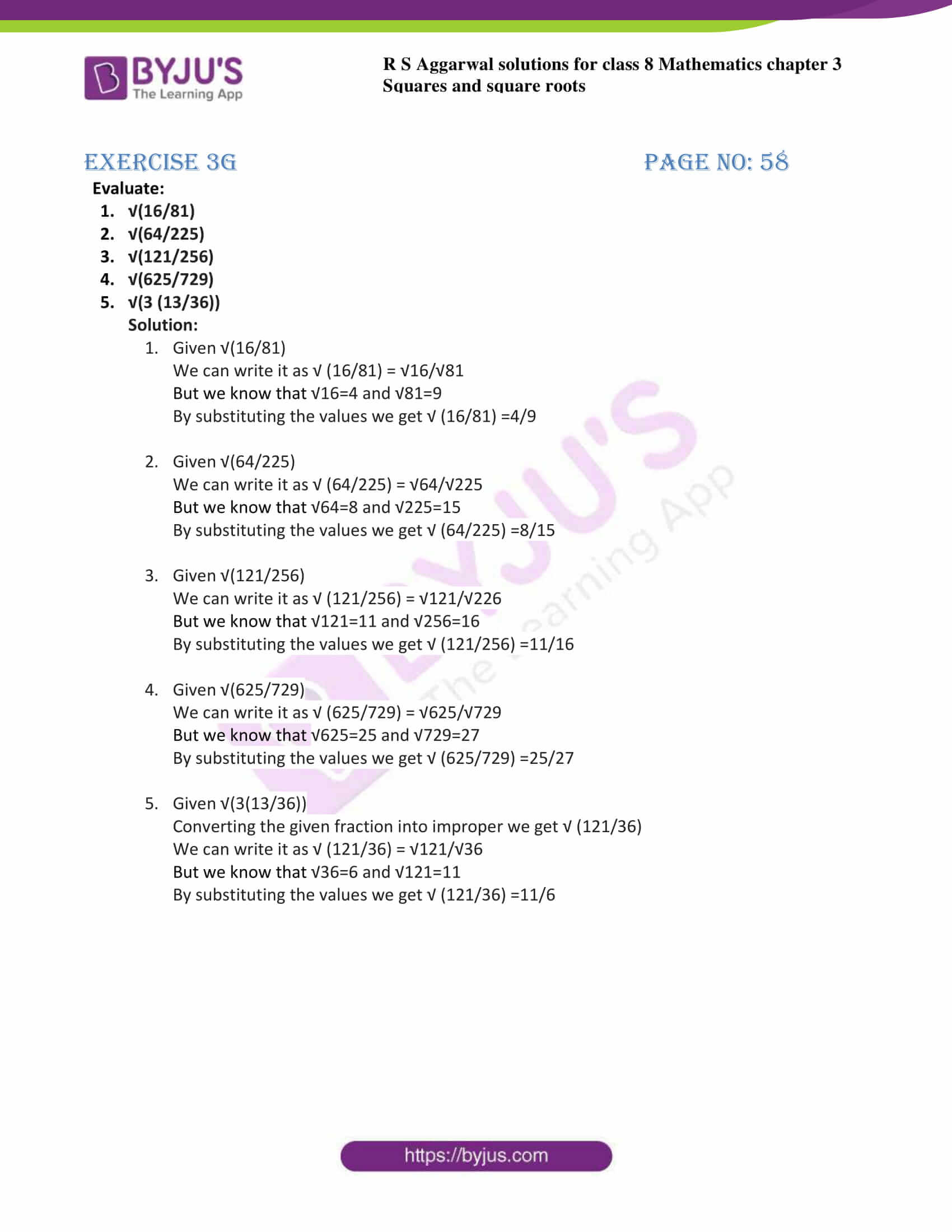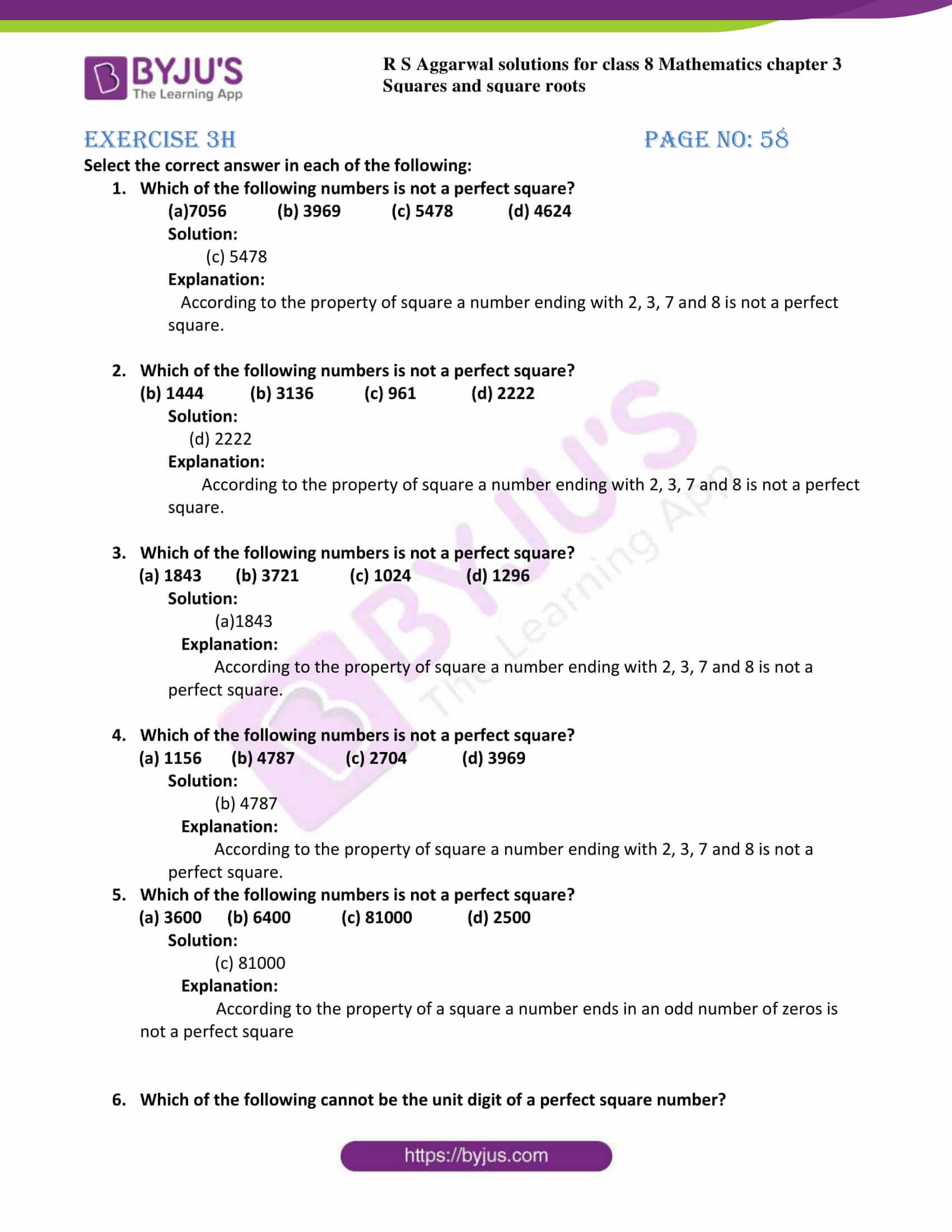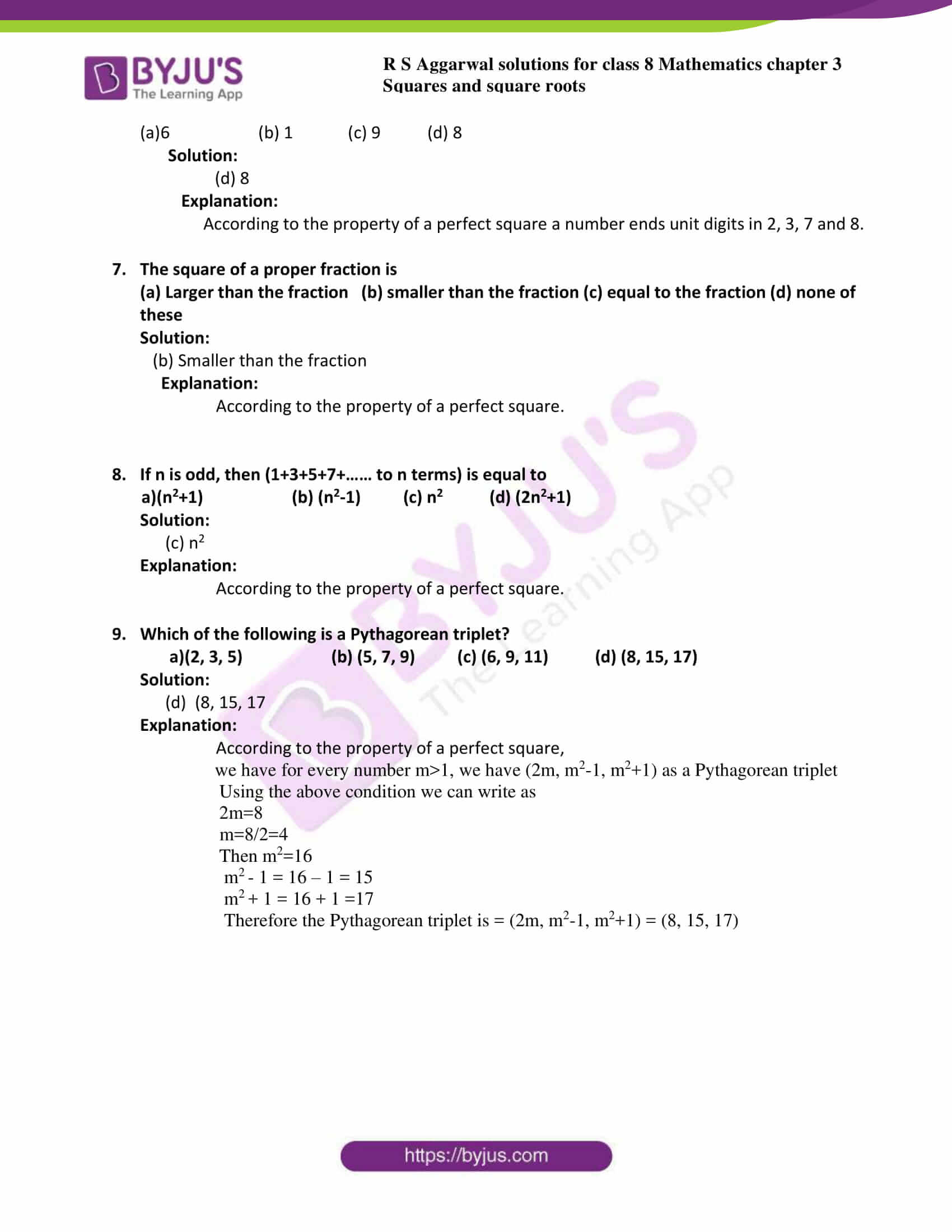## Exercise 3A

Q1. Using the prime factorization method, find which of the following numbers are perfect squares:

(i) 441

(ii) 576

(iii) 11025

(iv) 1176

(v) 5625

(vi) 9075

(vii) 4225

(viii) 1089

Solution:

1. Given 441, A perfect square can always be expressed as a product of pairs of equal factors.Now resolve 441 into prime factors, we get441 = 49 X 9 = 7 X 7 X 3 X 3 = 7 X 3 X 7 X 3 = 21 X 21 = (21)2Hence, 21 is the number whose square is 441

∴ 441 is a perfect square.

2. Given 576A perfect square can always be expressed as a product of pairs of equal factors.Now resolve 576 into prime factors, we get576 = 64 X 9 = 8 X 8 X 3 X 3 = 8 X 3 X 8 X 3 = 24 X 24 = (24)2Hence, 24 is the number whose square is 576

∴ 576 is a perfect square.

3. Given 11025A perfect square can always be expressed as a product of pairs of equal factors.Now resolve 11025 into prime factors, we get11025 = 441 X 25 = 49 X 9 = 7 X 7 X 3 X 3 X 5 X 5 = 7 X 3 X 5 X 7 X 3 X 5 = 105 X 105 = (105)2Hence, 105 is the number whose square is 11025

∴ 11025 is a perfect square.

4. Given 1176A perfect square can always be expressed as a product of pairs of equal factors.Now resolve 1176 into prime factors, we get1176 = 7 X 168Again resolve 168 into prime factors we get,

1176 = 7 X 168 = 7 X 21 X 8 = 7 X 7 X 3 X 2 X 2 X 2

1176 cannot be expressed as a product of two numbers.

Thus 1176 is not a perfect square.

5. Given 5625A perfect square can always be expressed as a product of pairs of equal factors.Now resolve 5625 into prime factors, we get5625 = 225 X 25 = 9 X 25 X 25 = 3 X 3 X 5 X 5 X 5 X 5 = 3 X 5 X 5 X 3 X 5 X 5= 75 X 75 = (75)2

Hence, 75 is the number whose square is 5625

∴ 5625 is a perfect square.

6. Given 9075A perfect square can always be expressed as a product of pairs of equal factors.Now resolve 9075 into prime factors, we get9075 = 25 X 363Again resolve 363 into prime factors we get,

9075 = 25 X 363 = 25 X 121 X 3 = 5 X 5 X 11 X 11 X 3 =5 X 11 X 5 X 11 X 3 = 55 X 55 X 3

1176 cannot be expressed as a product of two numbers.

Thus 1176 is not a perfect square.

7. Given 4225A perfect square can always be expressed as a product of pairs of equal factors.Now resolve 4225 into prime factors, we get4225 = 25 X 169 = 25 X 13 X 13 = 5 X 5 X 13 X 13 = 5 X 13 X 5 X 13= 65 X 65 = (65)2

Hence, 65 is the number whose square is 4225

∴ 4225 is a perfect square.

8. Given 1089A perfect square can always be expressed as a product of pairs of equal factors.Now resolve 1089 into prime factors, we get1089 = 9 X 121 = 3 X 3 X 11 X 11 = 3 X 11 X 3 X 11= 33 X 33 = (33)2

Hence, 33 is the number whose square is 1089

∴ 1089 is a perfect square.

Q2. Show that each of the following numbers is a perfect square. In each case, find the number whose square is the given number:

(i) 1225

(ii) 2601

(iii) 5929

(iv) 7056

(v) 8281

Solution:

1. Given 1225A perfect square can always be expressed as a product of pairs of equal factors.Now resolve 1225 into prime factors, we get1225 = 25 X 49 = 5 X 5 X 7 X 7 = 5 X 7 X 5 X 7 = 35 X 35 = (35)2Hence, 35 is the number whose square is 1225

∴ 1225 is a perfect square.

2. Given 2601A perfect square can always be expressed as a product of pairs of equal factors.Now resolve 2601 into prime factors, we get2601 = 9 X 289 = 3 X 3 X 17 X 17 = 3 X 17 X 3 X 17 = 51 X 51 = (51)2Hence, 51 is the number whose square is 2601

∴ 2601 is a perfect square.

3. Given 5929A perfect square can always be expressed as a product of pairs of equal factors.Now resolve 5929 into prime factors, we get5929 = 11 X 539 = 11 X 7 X 77 = 77 X 77 = (77)2Hence, 77 is the number whose square is 5929

∴ 5929 is a perfect square.

4. Given 7056A perfect square can always be expressed as a product of pairs of equal factors.Now resolve 7056 into prime factors, we get7056 = 11 X 539 = 12 X 588 = 12 X 7 X 84 = 84 X 84 = (84)2Hence, 84 is the number whose square is 5929

∴ 7056 is a perfect square.

5. Given 8281A perfect square can always be expressed as a product of pairs of equal factors.Now resolve 8281 into prime factors, we get8281 = 49 X 169 = 7 X 7 X 13 X 13 = 7 X 13 X 7 X 13 = 91 X 91 = (91)2Hence, 91 is the number whose square is 8281

∴ 8281 is a perfect square

Q3. By what least number should the given number be multiplied to get a perfect square number? In each case, find the number whose square is the new number.

(i) 3675

(ii) 2156

(iii) 3332

(iv) 2925

(v) 9075

(vi) 7623

(vii) 3380

(viii) 2475

Solution:

1. Given 3675Resolve 3675 into prime factors, we get3675 = 3 X 5 X 5 X 7 X 7Now to get perfect square we have to multiply the above equation by 3Then we get, 3675 = 3 X 3 X 5 X 5 X 7 X 7= (3 X 52 X 72)

New number = (9 X 25 X 49)

= (32 X 52 X 72)

Taking squares as common from the above equation we get

∴ New number = (3 X 5 X 7)2

= (105)2

Hence, the new number is square of 105

2. Given 2156Resolve 2156 into prime factors, we get2156 = 2 X 2 X 7 X 7 X 11= (22 X 72 X 11)Now to get perfect square we have to multiply the above equation by 11Then we get, 2156 = 2 X 2 X 7 X 7 X 11 X 11

New number = (4 X 49 X 121)

= (22 X 72 X 112)

Taking squares as common from the above equation we get

∴ New number = (2 X 7 X 11)2

= (154)2

Hence, the new number is square of 154

3. Given 3332Resolve 3332 into prime factors, we get3332 = 2 X 2 X 7 X 7 X 17= (22 X 72 X 17)Now to get perfect square we have to multiply the above equation by 17Then we get, 3332 = 2 X 2 X 7 X 7 X 17 X 17

New number = (4 X 49 X 289)

= (22 X 72 X 172)

Taking squares as common from the above equation we get

∴ New number = (2 X 7 X 17)2

= (238)2

Hence, the new number is square of 238

4. Given 2925Resolve 2925 into prime factors, we get2925 = 3 X 3 X 5 X 5 X 13= (32 X 52 X 13)Now to get perfect square we have to multiply the above equation by 13Then we get, 2156 = 3 X 3 X 5 X 5 X 13 X 13

New number = (9 X 25 X 169)

= (32 X 52 X 132)

Taking squares as common from the above equation we get

∴ New number = (3 X 5 X 13)2

= (195)2

Hence, the new number is square of 195

5. Given 9075Resolve 9075 into prime factors, we get9075 = 3 X 3 X 5 X 5 X 11= (32 X 52 X 11)Now to get perfect square we have to multiply the above equation by 11Then we get, 9075 = 3 X 3 X 5 X 5 X 11 X 11

New number = (9 X 25 X 121)

= (32 X 52 X 112)

Taking squares as common from the above equation we get

∴ New number = (3 X 5 X 11)2

= (165)2

Hence, the new number is square of 165

6. Given 7623Resolve 7623 into prime factors, we get7623= 3 X 3 X 7 X 11 X 11= (32 X 112 X 7)Now to get perfect square we have to multiply the above equation by 7Then we get, 9075 = 3 X 3 X 7 X 7 X 11 X 11

New number = (9 X 49 X 121)

= (32 X 72 X 112)

Taking squares as common from the above equation we get

∴ New number = (3 X 7 X 11)2

= (231)2

Hence, the new number is square of 231

7. Given 3380Resolve 3380 into prime factors, we get3380 = 2 X 2 X 5 X 13 X 13= (22 X 132 X 5)Now to get perfect square we have to multiply the above equation by 5Then we get, 3380 = 2 X 2 X 5 X 5 X 13 X 13

New number = (4 X 25 X 169)

= (22 X 52 X 132)

Taking squares as common from the above equation we get

∴ New number = (2 X 5 X 13)2

= (130)2

Hence, the new number is square of 130

8. Given 2475Resolve 2475 into prime factors, we get2475 = 3 X 3 X 5 X 5 X 11= (32 X 52 X 11)Now to get perfect square we have to multiply the above equation by 11Then we get, 3380 = 3 X 3 X 5 X 5 X 11 X 11

New number = (9 X 25 X 121)

= (32 X 52 X 112)

Taking squares as common from the above equation we get

∴ New number = (3 X 5 X 11)2

= (165)2

Hence, the new number is square of 165

4. By what least number should the given number be divided to get a perfect square number? In each case, find the number whose square is the new number

(i) 1575

(ii) 9075

(iii) 4851

(iv) 3380

(v) 4500

(vi) 7776

(vii) 8820

(viii) 4056

Solution:

1. Given 1575Resolve 1575 into prime factors, we get1575 = 3 X 3 X 5 X 5 X 7 = (32 X 52 X 7)Now to get perfect square we have to divide the above equation by 7Then we get, 3380 = 3 X 3 X 5 X 5

New number = (9 X 25)

= (32 X 52 )

Taking squares as common from the above equation we get

∴ New number = (3 X 5)2

= (15)2

Hence, the new number is square of 15

2. Given 9075Resolve 9075 into prime factors, we get9075 = 3 X 5 X 5 X 11 X 11 = (3 X 52 X 112)Now to get perfect square we have to divide the above equation by 3Then we get, 9075 = 5 X 5 X 11 X 11

New number = (25 X 121)

= (52 X 112 )

Taking squares as common from the above equation we get

∴ New number = (5 X 11)2

= (55)2

Hence, the new number is square of 55

3. Given 4851Resolve 4851 into prime factors, we get4851 = 3 X 3 X 7 X 7 X 11 = (32 X 72 X 11)Now to get perfect square we have to divide the above equation by11

Then we get, 9075 = 3 X 3 X 7 X 7

New number = (9 X 49)

= (32 X 72 )

Taking squares as common from the above equation we get

∴ New number = (3 X 7)2

= (21)2

Hence, the new number is square of 21

4. Given 3380Resolve 3380 into prime factors, we get3380 = 2 X 2 X 5 X 13 X 13 = (22 X 5 X 132)Now to get perfect square we have to divide the above equation by 5Then we get, 3380 = 2 X 2 X 13 X 13

New number = (4 X 169)

= (22 X 132 )

Taking squares as common from the above equation we get

∴ New number = (2 X 13)2

= (26)2

Hence, the new number is square of 26

5. Given 4500Resolve 4500 into prime factors, we get3380 = 2 X 2 X 3 X 3 X 5 X 5 X 5 = (22 X 3 2X 52 X 5)Now to get perfect square we have to divide the above equation by 5Then we get, 4500 = 2 X 2 X 3 X 3 X 5 X 5

New number = (4 X 9 X 25)

= (22 X 32 X 52 )

Taking squares as common from the above equation we get

∴ New number = (2 X 3 X 5)2

= (30)2

Hence, the new number is square of 30

6. Given 7776Resolve 7776 into prime factors, we get7776 = 2 X 2 X 2 X 2 X 2 X 3 X 3 X 3 X 3 X 3 = (22 X 2 2X 32 X 32 X 2 X 3)Now to get perfect square we have to divide the above equation by 2And 3

Then we get, 7776 = 2 X 2 X 2 X 2 X 3 X 3 X 3 X 3

New number = (4 X 4 X 9 X 9)

= (22 X 22 X 32 X 32)

Taking squares as common from the above equation we get

∴ New number = (2 X 2 X 3 X 3)2

= (36)2

Hence, the new number is square of 36

7. Given 8820Resolve 8820 into prime factors, we get8820 = 2 X 2 X 3 X 3 X 5 X 7 X 7 = (22 X 32 X 72 X 5)Now to get perfect square we have to divide the above equation by 5Then we get, 8820 = 2 X 2 X 3 X 3 X 7 X 7

New number = (4 X 9 X 49)

= (22 X 32 X 72)

Taking squares as common from the above equation we get

∴ New number = (2 X 2 X 3 X 3 X 7 X 7)2

= (42)2

Hence, the new number is square of 42

8. Given 4056Resolve 4056 into prime factors, we get4056 = 2 X 2 X 2 X 3 X 13 X 13 = (22 X 132 X 3 X 2)Now to get perfect square we have to divide the above equation by 3And 2

Then we get, 4056 = 2 X 2 X 13 X 13

New number = (4 X 169)

= (22 X 132)

Taking squares as common from the above equation we get

∴ New number = (2 X 2 X 13 X 13)2

= (26)2

Hence, the new number is square of 26

5. Find the largest number of 2 digits which is a perfect square.

Solution:

We know that the first three digit number is 100 and its square root is 10.

The number before 10 is 9

And we know the square of 9=92=81

Thus, the largest number of 2 digits is 81 which is a perfect square

6. Find the largest number of 3 digits which is a perfect square.

Solution:

We know that 3 digits largest number is 999.

We also know that the square root of 999 is 31.61

So the square of any number greater than 31.61 is 4 digit

Therefore, the square of 31 will be the largest number of 3 digits which is

Perfect square

## Exercise 3B

Q1. Give a reason to show that none of the numbers given below is a perfect square:

(i) 5372

(ii) 5963

(iii) 8457

(iv) 9468

(v) 360

(vi) 64000

(vii) 2500000

Solution:

1. Given 5372By observing the property 1 of perfect square i.e. a number ending in 2 is never a perfect square.Therefore the given number 5372 is not a perfect square.
2. Given 5963We have the property 1 of perfect square i.e. a number ending in 3 is never a perfect square.Therefore the given number 5963 is not a perfect square.
3. Given 8457We have the property 1 of perfect square i.e. a number ending in 7 is never a perfect square.Therefore the given number 8457 is not a perfect square.
4. Given 9468We have the property 1 of perfect square i.e. a number ending in 8 is never a perfect square.Therefore the given number 9458 is not a perfect square.
5. Given 360We know the property 2 of perfect square i.e. a number ending in an add number of zeros is never a perfect square.Therefore the given number 360 is not a perfect square.
6. Given 64000We know the property 2 of perfect square i.e. a number ending in an add number of zeros is never a perfect square.Therefore the given number 64000 is not a perfect square.
7. Given 2500000We know the property 2 of perfect square i.e. a number ending in an add number of zeros is never a perfect square.Therefore the given number 2500000 is not a perfect square.

Q2. Which of the following are squares of even numbers?

(i) 196

(ii) 441

(iii) 900

(iv) 625

(v) 324

Solution:

1. Given 196We know that from the property of perfect square, the square of an even number is always even.So the given number 196 is even, therefore it is square of even number
2. Given 441We know that from the property of perfect square, the square of an even number is always even.But the given number 441 is odd, therefore it is not a square of even number
3. Given 900We know that from the property of perfect square, the square of an even number is always even.So the given number 900 is even, therefore it is square of even number
4. Given 625We know that from the property of perfect square, the square of an even number is always even.But the given number 625 is odd, therefore it is not a square of even number
5. Given 324We know that from the property of perfect square, the square of an even number is always even.So the given number 324 is even, therefore it is square of even number

Q3. Which of the following are squares of add numbers?

(i) 484

(ii) 961

(iii) 7396

(iv) 8649

(v) 4225

Solution:

1. Given 484 According to the property of perfect square i.e. the square of a odd number is always odd.But the given number 484 is not oddTherefore it is not square of odd number.
2. Given 961According to the property of perfect square i.e. the square of a odd number is always odd.The given number 961 is odd, therefore it is square of odd number.
3. Given 7396According to the property of perfect square i.e. the square of a odd number is always odd.But the given number 7396 is not oddTherefore it is not square of odd number.
4. Given 8649According to the property of perfect square i.e. the square of an odd number is always odd.The given number 8649 is odd, therefore it is square of odd number.
5. Given 4225According to the property of perfect square i.e. the square of an odd number is always odd.The given number 4225 is odd, therefore it is square of odd number.

Q4. Without adding, find the sum:

(i) (1+3+5+7+9+11+13)

(ii) (1+3+5+7+9+11+13+15+17+19)

(iii) (1+3+5+7+9+11+13+15+17+19+21+23)

Solution:

1. Given (1+3+5+7+9+11+13)According to the property of perfect square, for any natural number n, we have sum of first n odd natural numbers=n2But here n=7Applying the above law we getTherefore (1+3+5+7+9+11+13) = 72 = 49
2. Given (1+3+5+7+9+11+13+15+17+19)According to the property of perfect square, for any natural number n, we have sum of first n odd natural numbers=n2But here n=10Applying the above law we getTherefore (1+3+5+7+9+11+13+15+17+19) = 102 = 100
3. Given (1+3+5+7+9+11+13+15+17+19+21+23)According to the property of perfect square, for any natural number n, we have the sum of first n odd natural numbers=n2But here n=12Applying the above law we getTherefore (1+3+5+7+9+11+13+15+17+19+21+23) = 122 = 144

Q5.

(i) Express 81 as the sum of 9 odd numbers.

(ii) Express 100 as the sum of 10 odd numbers.

Solution:

1. Given 81 can be written as 92According to the property of perfect square, for any natural number n, we have sum of first n odd natural numbers=n2Expressing 81 as a sum of 9 odd numbers, we get81= (9)2Here n=9

By applying the law 81 = (1+3+5+7+9+11+13+15+17)

2. Given 100 can be written as 102According to the property of perfect square, for any natural number n, we have sum of first n odd natural numbers=n2Expressing 100 as a sum of 10 odd numbers, we get100 = (10)2Here n=10

By applying the law 81 = (1+3+5+7+9+11+13+15+17+19)

Q6. Write a Pythagorean triplet whose smallest member is

(i) 6

(ii) 14

(iii) 16

(iv) 20

Solution:

1. Given 6 is the smallest number in Pythagorean tripletBut we have for every number m>1, we have (2m, m2-1, m2+1) as a Pythagorean tripletUsing the above condition we can write as2m=6m=6/2=3

Then m2=9

m2 – 1 = 9 – 1 = 8

m2 + 1 = 9 + 1 =10

Therefore the Pythagorean triplet is = (2m, m2-1, m2+1) = (6, 8, 10)

2. Given 14 is the smallest number in Pythagorean tripletBut we have for every number m>1, we have (2m, m2-1, m2+1) as a Pythagorean tripletUsing the above condition we can write as2m=14m= 14/2=7

Then m2 =49

m2 – 1 = 49 – 1 = 48

m2 + 1 = 49 + 1 =50

Therefore the Pythagorean triplet is = (2m, m2-1, m2+1) = (14, 48, 50)

3. Given 16 is the smallest number in Pythagorean tripletBut we have for every number m>1, we have (2m, m2-1, m2+1) as a Pythagorean tripletUsing the above condition we can write as2m=16m= 16/2=8

Then m2 =64

m2 – 1 = 64 – 1 = 63

m2 + 1 = 64 + 1 =65

Therefore the Pythagorean triplet is = (2m, m2-1, m2+1) = (16, 63, 65)

4. Given 20 is the smallest number in Pythagorean tripletBut we have for every number m>1, we have (2m, m2-1, m2+1) as a Pythagorean tripletUsing the above condition we can write as2m=20m= 20/2=10

Then m2 =100

m2 – 1 = 100 – 1 = 99

m2 + 1 = 100 + 1 =101

Therefore the Pythagorean triplet is = (2m, m2-1, m2+1) = (20, 99, 101)

Q7. Evaluate:

(i) (38)2 –(37)2

(ii) (75)2 –(74)2

(iii) (92)2 –(91)2

(iv) (105)2 –(104)2

(v) (141)2 –(140)2

(vi) (218)2 –(217)2

Solution:

1. Given (38)2 –(37)2According to the property 8 i.e. for every natural number n, we have [(n+1)2-n2] = [(n+1) + n]Here n=37By applying the above property we get,(38)2 – (37)2 = (37+1) + 37 = 38+ 37 = 75
2. Given (75)2 –(74)2According to the property 8 i.e. for every natural number n, we have [(n+1)2-n2] = [(n+1) + n]Here n=74By applying the above property we get,(75)2 – (74)2 = (74+1) + 74 = 75 + 74 = 149
3. Given (92)2 –(91)2According to the property 8 i.e. for every natural number n, we have [(n+1)2-n2] = [(n+1) + n]Here n=91By applying the above property we get,(92)2 – (91)2 = (91+1) + 91 = 92 + 91 = 183
4. Given (105)2 –(104)2According to the property 8 i.e. for every natural number n, we have [(n+1)2-n2] = [(n+1) + n]Here n=104By applying the above property we get,(105)2 – (104)2 = (104+1) + 104 = 105 + 104 = 209
5. Given (141)2 –(140)2According to the property 8 i.e. for every natural number n, we have [(n+1)2-n2] = [(n+1) + n]Here n=140By applying the above property we get,(141)2 – (140)2 = (140+1) + 140 = 141 + 140 = 281
6. Given (218)2 –(217)2According to the property 8 i.e. for every natural number n, we have [(n+1)2-n2] = [(n+1) + n]Here n=217By applying the above property we get,= – (217)2 = (217+1) + 217 = 218 + 217 = 435

Q8. Using the formula (a + b)2=(a2 + 2ab + b2), evaluate:

(i) (310)2

(ii) (508)2

(iii) (630)2

Solution:

1. Given (310)2We have (a + b) 2 = (a2 + 2ab + b2)(310)2 can be written as 300+10So here a=300 and b=10Using the formula,

(300 + 10) 2 = (3002 + 2 X 300 X 10 + 102)

On simplifying we get

(300 + 10) 2 = (90000 + 6000 +100)

(310) 2=96100

2. Given (508)2We have (a + b) 2 = (a2 + 2ab + b2)(508)2 can be written as 500+8So here a=500 and b=8Using the formula,

(500 + 8) 2 = (5002 + 2 X 500 X 8 + 82)

On simplifying we get

(500 + 8) 2 = (250000 + 8000 +64)

(508) 2 = 258064

3. Given (630)2We have (a + b) 2 = (a2 + 2ab + b2)(630)2 can be written as 600+30So here a=300 and b=10Using the formula,

(600 + 30) 2 = (6002 + 2 X 600 X 30 + 302)

On simplifying we get,

(600 + 30) 2 = (360000 + 3600 +900)

(630) 2 = 396900

Q9. Using the formula (a – b)2=(a2 – 2ab + b2), evaluate:

(i) (196)2

(ii) (689) 2

(iii) (891) 2

Solution:

1. Given (196)2We have (a – b) 2 = (a2 – 2ab + b2)(196)2 can be written as 200-4So here a=200 and b=4Using the formula,

(200 – 4) 2 = (2002 – 2 X 200 X 4 + 42)

On simplifying we get

(200 – 4) 2 = (40000 – 1600 +16)

(196) 2=38416

2. Given (689)2We have (a – b) 2 = (a2 – 2ab + b2)(689)2 can be written as 700-11So here a=700 and b=11Using the formula,

(700 – 11) 2 = (7002 – 2 X 700 X 11 + 112)

On simplifying we get

(700 – 11) 2 = (490000 – 15400 +121)

(689) 2=474721

3. Given (891)2We have (a – b) 2 = (a2 – 2ab + b2)(891)2 can be written as 900-9So here a=900 and b=9Using the formula,

(900 – 9) 2 = (9002 – 2 X 900 X 9 + 92)

On simplifying we get

(900 – 9) 2 = (810000 – 16200 +81)

(891) 2=793881

Q10. Evaluate:

(i) 69 X 71

(ii) 94 X 106

Solution:

1. Given 69 X 71We can write 69 as 70-1 and also 71 as 70+1We know that (a + b) X (a – b) = a2– b2Here a= 70 and b = 1Applying the above formula we get

(70 + 1) X (70 – 1) = (70)2 – (1)2

(70 + 1) X (70 – 1) = 4900 – 1= 4899

2. Given 94 X 106We can write 94 as 100-6 and also 106 as 100+6We know that (a + b) X (a – b) = a2– b2Here a= 100 and b = 6Applying the above formula we get

(100 + 6) X (100 – 6) = (100)2 – (6)2

(100 + 6) X (100 – 6) = 10000 – 36= 9964

Q11. Evaluate:

(i) 88 X 92

(ii) 78 X 82

Solution:

1. Given 88 X 92We can write 88 as 90-2 and also 92 as 90+2We know that (a + b) × (a – b) = a2– b2Here a= 90 and b = 2Applying the above formula we get

(90 + 2) X (90 – 2) = (90)2 – (2)2

(90 + 2) X (90 – 2) = 8100 – 4= 8096

2. Given 78 X 82We can write 78 as 80-2 and also 82 as 80+2We know that (a + b) X (a – b) = a2– b2Here a= 80 and b = 2Applying the above formula we get

(80 + 2) X (80 – 2) = (80)2 – (2)2

(80 + 2) X (80 – 2) = 6400 – 4= 6396

Q12. Fill in the blanks:

(i) The square of an even number is……….

(ii) The square of an odd number is…………

(iii) The square of a proper fraction is………. than the given fraction.

(iv) n2= the sum of first n ……… natural numbers.

Solution:

1. Even Explanation: According to the property of perfect square i.e. the square of anEven number is always even.
2. Odd Explanation: According to the property of perfect square i.e. the square of an odd number is always odd.
3. SmallerExplanation: According to the property of perfect square i.e. the square of a proper fraction is smaller than the fraction.
4. OddExplanation: According to the property of perfect square i.e. for ever natural number n, we have sum of first n odd natural numbers = n2

Q13. Write (T) for true and (F) for false for each of the statements given below:

(i) The number of digits in a perfect square is even.

(ii) The square of a prime number is prime.

(iii) The sum of two perfect squares is a perfect squares.

(iv) The difference of two perfect squares is a perfect square.

(v) The product of two perfect squares is a perfect squares.

1. Solution:FalseExplanation:Because the number of digits present in perfect square also be odd.
2. Solution: FalseExplanation: A prime number is one that is not divisible by any other, except by 1 and itself. So square of a prime number is not always prime.
3. Solution: FalseExplanation: The sum of two perfect square can be any number.
4. Solution: FalseExplanation: The difference of two perfect square can be any number.
5. Solution: TrueExplanation: According to the properties of perfect square we have,The product of two perfect squares is a perfect square.

## Exercise 3C

Find the value of each of the following, using the column method:

1. (23)2

2. (35)2

3. (52)2

4. (96)2

Solution:

1. Given number = 23By using column method we have,∴ a=2 and b=3
 a2 (2 × a × b) b2 4 + 1 =5 12 +0 =12 9

∴ (23)2=529

Steps involved in solving column method:

Step 1: Make three columns headed by a2, 2 X a x b and b2 respectively. Write the values of a2, 2 X a x b and b2 in columns respectively.

Step 2: In third column underline the unit digits of b2 i.e. 9 and carry the tens digit of it i.e. 0 to the second column and add it to the value of second column i.e. 2 X a x b and it will remains 12 if it added to 0.

Step 3: In column second, underline the unit digit of the number obtained in second step i.e. 2 and carry over the ten digit of it to first column and add it to the value of a2 i.e. 4+1=5

Step 4: Now underline the number obtained in third step in first column i.e. 5. The underlined digits give the required square number.

2. Given number = 35By using column method we have,∴ a=3 and b=5
 a2 (2 × a × b) b2 9 + 3 =12 30 +2 =32 25

∴ (35)2=1225

Steps involved in solving column method:

Step 1: Make three columns headed by a2, 2 X a x b and b2 respectively. Write the values of a2, 2 × a × b and b2 in columns respectively.

Step 2: In third column underline the unit digits of b2 i.e. 9 and carry the tens digit of it i.e. 0 to the second column and add it to the value of second column i.e. 2 × a × b and it will becomes 25 and underline 5.

Step 3: In column second, underline the unit digit of the number obtained in second step i.e. 2 and carry over the ten digit of it to first column and add it to the value of a2 i.e. 30+2=32

Step 4: Now underline the number obtained in third step in first column i.e. 12. The underlined digits give the required square number.

3. Given number = 52By using column method we have,∴ a=5 and b=2
 a2 (2 × a × b) b2 25 + 2 =27 20 +0 =20 4

∴ (52)2=2704

Steps involved in solving column method:

Step 1: Make three columns headed by a2, 2 × a × b and b2 respectively. Write the values of a2, 2 × a × b and b2 in columns respectively.

Step 2: In third column underline the unit digits of b2 i.e. 4 and carry the tens digit of it i.e. 0 to the second column and add it to the value of second column i.e. 2 × a × b and it will remains 20 if it added to 0.

Step 3: In column second, underline the unit digit of the number obtained in second step i.e. 0 and carry over the ten digit of it to first column and add it to the value of a2 i.e. 25+2=27

Step 4: Now underline the number obtained in third step in first column i.e. 27. The underlined digits give the required square number.

4. Given number = 96By using column method we have,∴ a=9 and b=6
 a2 (2 × a × b) b2 81 + 11 =92 108 +3 =111 36

∴ (96)2=9216

Steps involved in solving column method:

Step 1: Make three columns headed by a2, 2 x a x b and b2 respectively. Write the values of a2, 2 × a × b and b2 in columns respectively.

Step 2: In third column underline the unit digits of b2 i.e. 36 and carry the tens digit of it i.e. 3 to the second column and add it to the value of second column i.e. 2 × a × b and it will becomes 111.

Step 3: In column second, underline the unit digit of the number obtained in second step i.e. 1 and carry over the ten digit of it to first column and add it to the value of a2 i.e. 81+11=92

Step 4: Now underline the number obtained in third step in first column i.e. 92. The underlined digits give the required square number.

Find the value of each of the following, using the diagonal method.

5. (67)2

6. (86)2

7. (137)2

8. (256)2

1. Solutions: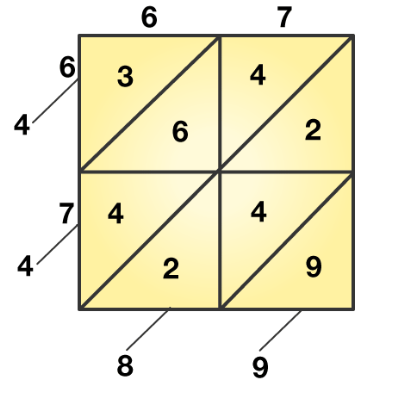Given number = 67Steps involved in solving diagonal method:Step 1: The given number contains two digits. So, draw a square and divide it into 4 sub squares as shown above. Write down the digits 6 and 7 vertically, as shown in above.

Step 2: Multiply each digit on the left of the square with each digit on the top, one by one. Write the product in the corresponding subsequence.

If the product is a two-digit number, write the tens digit above the diagonal and the units digit below the diagonal.

Step 3: Starting below the lowest diagonal, sum the digits diagonally.

If the sum is a two-digit number, underline the unit digit and carry over the tens digit to the next diagonal.

Step 4: underline all the digits in the sum above the topmost diagonal.

Step 5: the underlined digits give the required square number.

2. Solutions: Given number = 86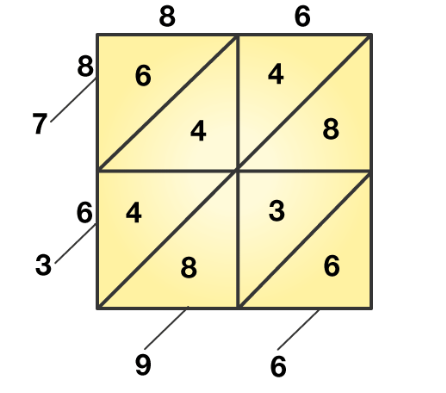Steps involved in solving diagonal method:Step 1: The given number contains two digits. So, draw a square and divide it into 4 sub squares as shown above. Write down the digits 6 and 7 vertically, as shown in above.Step 2: Multiply each digit on the left of the square with each digit on the top, one by one. Write the product in the corresponding subsequence.

If the product is a two-digit number, write the tens digit above the diagonal and the units digit below the diagonal.

Step 3: Starting below the lowest diagonal, sum the digits diagonally.

If the sum is a two-digit number, underline the unit digit and carry over the tens digit to the next diagonal.

Step 4: underline all the digits in the sum above the topmost diagonal.

Step 5: the underlined digits give the required square number.

3. Solutions: Given number = 137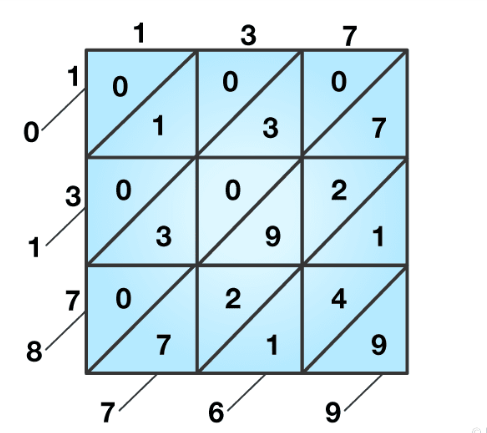Steps involved in solving diagonal method:Step 1: The given number contains two digits. So, draw a square and divide it into 4 sub squares as shown above. Write down the digits 6 and 7 vertically, as shown in above.

Step 2: Multiply each digit on the left of the square with each digit on the top, one by one. Write the product in the corresponding subsequence.

If the product is a two-digit number, write the tens digit above the diagonal and the units digit below the diagonal.

Step 3: Starting below the lowest diagonal, sum the digits diagonally.

If the sum is a two-digit number, underline the unit digit and carry over the tens digit to the next diagonal.

Step 4: underline all the digits in the sum above the topmost diagonal.

Step 5: the underlined digits give the required square number.

4. Solutions: Given number = 256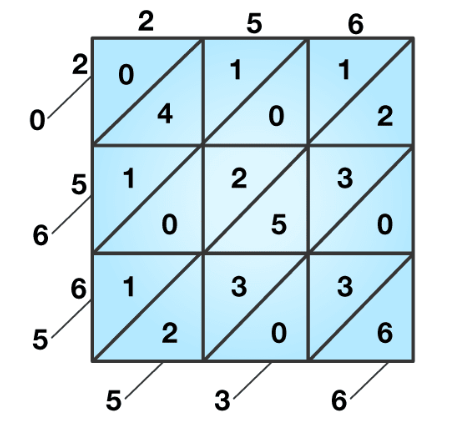Steps involved in solving diagonal method:Step 1: The given number contains two digits. So, draw a square and divide it into 4 sub squares as shown above. Write down the digits 6 and 7 vertically, as shown in above.

Step 2: Multiply each digit on the left of the square with each digit on the top, one by one. Write the product in the corresponding subsequence.

If the product is a two-digit number, write the tens digit above the diagonal and the units digit below the diagonal.

Step 3: Starting below the lowest diagonal, sum the digits diagonally.

If the sum is a two-digit number, underline the unit digit and carry over the tens digit to the next diagonal.

Step 4: underline all the digits in the sum above the topmost diagonal.

Step 5: the underlined digits give the required square number.

## Exercise 3D

1. Find the square root of each of the following numbers by using the method of prime factorization:

1. 225
2. 441
3. 729
4. 1296
5. 2025
6. 4096
7. 7056
8. 8100
9. 9216
10. 11025
11. 15876
12. 17424
1. Solution: Given 225A square can always be expressed as a product of pairs of equal factorsResolve the given number into prime factorsWe get 225=3 × 3 × 5 × 5

√225=3 × 5 = 15

2. Solution: Given 441A square can always be expressed as a product of pairs of equal factorsResolve the given number into prime factorsWe get 441=3 × 3 × 7 × 7

√441 = 3 × 7 = 21

3. Solution: Given 729A square can always be expressed as a product of pairs of equal factorsResolve the given number into prime factorsWe get 729 =3 × 3 × 3 × 3 × 3 × 3

√729=3 × 3 × 3 = 27

4. Solution: Given 1296A square can always be expressed as a product of pairs of equal factorsResolve the given number into prime factorsWe get 1296 =2 × 2 × 2 × 2 × 3 × 3 × 3 × 3

√1296=2 × 2 × 3 × 3 = 36

5. Solution: Given 2025A square can always be expressed as a product of pairs of equal factorsResolve the given number into prime factorsWe get 1296 =3 × 3 × 3 × 3 × 5 × 5

√2025=3 × 3 × 5 = 45

6. Solution: Given 4096A square can always be expressed as a product of pairs of equal factorsResolve the given number into prime factorsWe get 4096 =2 × 2 × 2 × 2 × 2 × 2 × 2 × 2 × 2 × 2 × 2 × 2

√4096=2 × 2 × 2 × 2 × 2 × 2 = 64

7. Solution: Given 7056A square can always be expressed as a product of pairs of equal factorsResolve the given number into prime factorsWe get 7056 =2 × 2 × 2 × 2 × 3 × 3 × 7 × 7

√7056=2 × 2 × 3 × 7 = 84

8. Solution: Given 8100A square can always be expressed as a product of pairs of equal factorsResolve the given number into prime factorsWe get 8100 =2 × 2 × 3 × 3 × 3 × 3 × 5 × 5

√8100=2 × 3 × 3 × 5 = 90

9. Solution: Given 9216A square can always be expressed as a product of pairs of equal factorsResolve the given number into prime factorsWe get 9216 =2 × 2 × 2 × 2 × 2 × 2 × 2 × 2 × 2 × 2 × 3 × 3

√9216 = 2 × 2 × 2 × 2 × 2 × 3 = 96

10. Solution: Given 11025A square can always be expressed as a product of pairs of equal factorsResolve the given number into prime factorsWe get 11025 =3 × 3 × 5 × 5 × 7 × 7

√11025 = 3 × 5 × 7 = 105

11. Solution:Given 15876A square can always be expressed as a product of pairs of equal factorsResolve the given number into prime factorsWe get 15876 =2 × 2 × 3 × 3 × 3 × 3 × 7 × 7

√15876 = 2 × 3 × 3 × 7= 126

12. Solution:Given 17424A square can always be expressed as a product of pairs of equal factorsResolve the given number into prime factorsWe get 17424 =2 × 2 × 2 × 2 × 3 × 3 × 11 × 11

√15876 = 2 × 2 × 3 × 11= 132

13. Find the smallest number by which 252 must be multiplied to get a perfect square. Also, find the square root of the perfect square so obtained. Solution:Given number= 252Resolve the given number into prime factors we get252=2 × 2 × 3 × 3 × 7

To get perfect square we have to multiply by 7

Then we get 252=2 × 2 × 3 × 3 × 7 × 7

New number=252 × 7 = 1764

Therefore √1764 = 2 × 3 × 7=42

2. Find the smallest number by which 2925 must be multiplied to get a perfect square. Also, find the square root of the perfect square so obtained.

Solution:

Given number= 2925

Resolve the given number into prime factors we get

2925=3 × 3 × 5 × 5 × 13

To get perfect square we have to divide by 13

Then we get 2925= 3 × 3 × 5 × 5

New number = 2925 / 13 = 225

Therefore √225 = 3 × 5= 15

3. 1225 plants are to be planted in a garden in such a way that each row contains as many plants as the number of rows. Find the number of rows and the number of plants in each row.

Solution:

Given: the number of plants to be planted = 1225

Let the number of rows be ‘x’

Therefore, the number plants in each row is also ‘x’

Total number of plants = (x × x) = x2=1225

Shifting the square, we get

√1225 = 5 × 7 = 35

Therefore, the number of rows id 35 and number of plants in each row is also 35

4. The students of a class arranged in a picnic. Each student contributed as many rupees as the number of students in the class. If the total contribution is Rs. 1156, find the strength of the class.

Solution:

Given: total contribution from students= Rs. 1156

Let the number of students be ‘x’

And also let the amount contributed by each student be ‘x’

Therefore, total amount contributed by the students = (x × x) = x2=1156

Shifting the square, we get

√1156 = 2 × 2 × 17 × 17 = 17 × 2 =34

Therefore, number of students is 43 and amount contributed by each student is also 34

5. Find the least square number which is exactly divisible by each of the numbers 6, 9, 15 and 20.

Solution:

Given numbers = 6, 9, 15 and 20

The smallest number divisible by 6, 9, 15 and 20 is their L.C.M. i.e. 180

Resolving the L.C.M. as prime factors we get, 180= 2 × 2 × 3 × 3 × 5

To make it perfect square multiply by 5 then it becomes, 180= 2 × 2 × 3 × 3 × 5 × 5

Which implies 180 × 5 = 900

Least square number which is exactly divisible by 6, 9, 15 and 20 is 900.

6. Find the least square number which is exactly divisible by each of the numbers 8, 12, 15 and 20.

Solution:

Given numbers = 8, 12, 15 and 20

The smallest number divisible by 8, 12, 15 and 20 is their L.C.M. i.e. 120

Resolving the L.C.M. as prime factors we get, 120= 2 × 2 × 2 × 3 × 5

To make it perfect square multiply by 2 × 3 × 5=30

Which implies 120 × 30 = 3600

Least square number which is exactly divisible by 6, 9, 15 and 20 is 3600.

## Exercise 3E

1. Evaluate:

1. √576
2. √1444
3. √4489
4. √6241
5. √7056
6. √9025
7. √11449
8. √14161
9. √10404

Solution: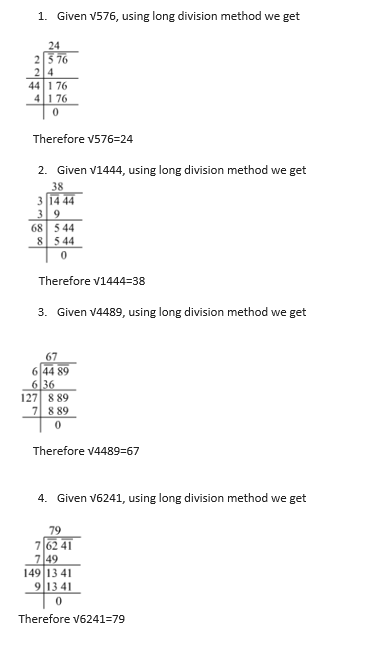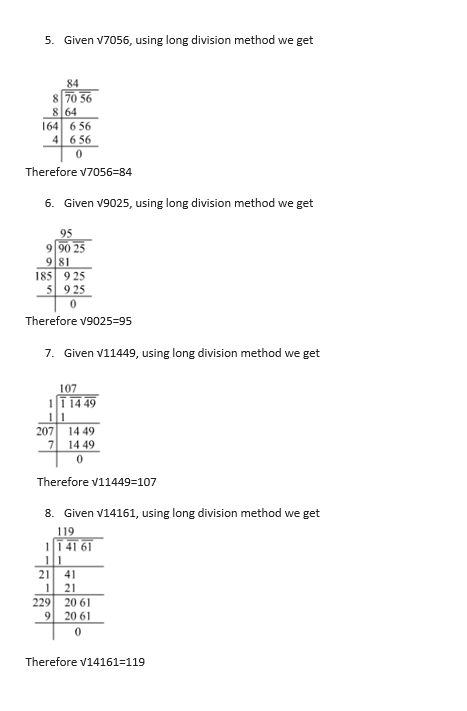9. Given √14161, using long division method we get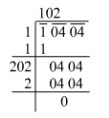Therefore √10404 = 102

## Exercise 3F

1. Evaluate:

1. √1.69
2. √33.64
3. √156.25
4. √75.69
5. √9.8596
6. √10.0489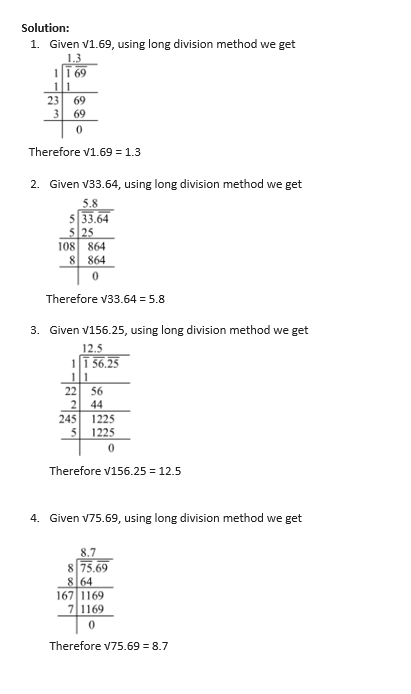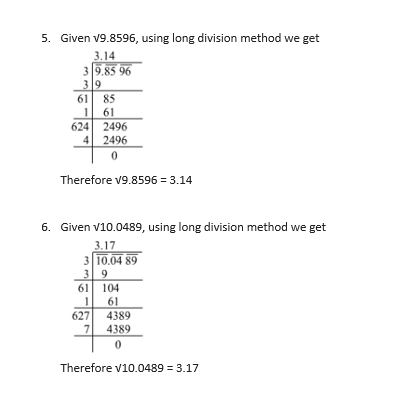## Exercise 3G

1. Evaluate:

1. √(16/81)
2. √(64/225)
3. √(121/256)
4. √(625/729)
5. √(3 (13/36))

Solution:

1. Given √(16/81)We can write it as √ (16/81) = √16/√81But we know that √16=4 and √81=9By substituting the values we get √ (16/81) =4/9
2. Given √(64/225)We can write it as √ (64/225) = √64/√225But we know that √64=8 and √225=15By substituting the values we get √ (64/225) =8/15
3. Given √(121/256)We can write it as √ (121/256) = √121/√226But we know that √121=11 and √256=16By substituting the values we get √ (121/256) =11/16
4. Given √(625/729)We can write it as √ (625/729) = √625/√729But we know that √625=25 and √729=27By substituting the values we get √ (625/729) =25/27
5. Given √(3(13/36))Converting the given fraction into improper we get √ (121/36)We can write it as √ (121/36) = √121/√36But we know that √36=6 and √121=11By substituting the values we get √ (121/36) =11/6

## Exercise 3H

1.Select the correct answer in each of the following:

1. Which of the following numbers is not a perfect square?(a)7056 (b) 3969 (c) 5478 (d) 4624Solution: (c) 5478Explanation:

According to the property of square a number ending with 2, 3, 7 and 8 is not a perfect square.

2. Which of the following numbers is not a perfect square?(b) 1444 (b) 3136 (c) 961 (d) 2222Solution: (d) 2222Explanation:

According to the property of square a number ending with 2, 3, 7 and 8 is not a perfect square.

3. Which of the following numbers is not a perfect square? (a) 1843 (b) 3721 (c) 1024 (d) 1296Solution: (a)1843Explanation:

According to the property of square a number ending with 2, 3, 7 and 8 is not a perfect square.

4. Which of the following numbers is not a perfect square? (a) 1156 (b) 4787 (c) 2704 (d) 3969Solution: (b) 4787Explanation:

According to the property of square a number ending with 2, 3, 7 and 8 is not a perfect square.

5. Which of the following numbers is not a perfect square? (a) 3600 (b) 6400 (c) 81000 (d) 2500Solution: (c) 81000Explanation:

According to the property of a square a number ends in an odd number of zeros is not a perfect square

6. Which of the following cannot be the unit digit of a perfect square number?(a)6 (b) 1 (c) 9 (d) 8Solution: (d) 8Explanation:

According to the property of a perfect square a number ends unit digits in 2, 3, 7 and 8.

7. The square of a proper fraction is (a) Larger than the fraction (b) smaller than the fraction (c) equal to the fraction (d) none of these Solution: (b) Smaller than the fractionExplanation:

According to the property of a perfect square.

8. If n is odd, then (1+3+5+7+…… to n terms) is equal to a)(n2+1) (b) (n2-1) (c) n2 (d) (2n2+1) Solution: (c) n2Explanation:

According to the property of a perfect square.

9. Which of the following is a Pythagorean triplet? a)(2, 3, 5) (b) (5, 7, 9) (c) (6, 9, 11) (d) (8, 15, 17) Solution: (d) (8, 15, 17Explanation:

According to the property of a perfect square,

we have for every number m>1, we have (2m, m2-1, m2+1) as a Pythagorean triplet

Using the above condition we can write as

2m=8

m=8/2=4

Then m2=16

m2 – 1 = 16 – 1 = 15

m2 + 1 = 16 + 1 =17

Therefore the Pythagorean triplet is = (2m, m2-1, m2+1) = (8, 15, 17)

## RS Aggarwal Solutions for Class 8 Maths Chapter 3- Squares and Square Roots

Chapter 3, Squares and Square Roots, contains 8 Exercises. RS Aggarwal Solutions given here includes the answers to all the questions present in these exercises. Let us have a look at some of the concepts that are being discussed in this chapter.

• Perfect Square or Square numbers
• Properties of Perfect Squares
• Product of two consecutive odd or consecutive even numbers
• Column method for squaring two-digit numbers.
• Diagonal method for squaring a number
• Definition of square roots
• Square root of a perfect square by the prime factorization method
• Square root of a perfect square by the long division method
• Square roots of a number in decimal form
• Square root of fractions

Exercise 3A

Exercise 3B

Exercise 3C

Exercise 3D

Exercise 3E

Exercise 3F

Exercise 3G

Exercise 3H

### Chapter Brief of RS Aggarwal Solutions for Class 8 Maths Chapter 3 – Squares and Square roots

The RS Aggarwal Solutions for Class 8 Maths Chapter 3 – Squares and Square Roots deals with the properties of perfect squares which in turn help to solve problems easily and quickly. By learning these concepts thoroughly we can find squares and square roots of given number without using the calculator.